# Forward Gravity Modelling to Augment High-Resolution Combined Gravity Field Models

## Abstract

During the last few years, the determination of high-resolution global gravity field has gained momentum due to high-accuracy satellite-derived observations and development of forward gravity modelling. Forward modelling computes the global gravitational field from mass distribution sources instead of actual gravity measurements and helps improving and complementing the medium to high-frequency components of the global gravity field models. In this study, we approximate the global gravity potential of the Earth’s upper crust based on ellipsoidal approximation and a mass layer concept. Such an approach has an advantage of spectral methods and also avoids possible instabilities due to the use of a sequence of thin ellipsoidal shells. Lateral density within these volumetric shells bounded by confocal lower and upper shell ellipsoids is used in the computation of the ellipsoidal harmonic coefficients which are then transformed into spherical harmonic coefficients on the Earth’s surface in the final step. The main outcome of this research is a spectral representation of the gravitatioal potential of the Earth’s upper crust, computed up to degree and order 3660 in terms of spherical harmonic coefficients (ROLI_EllApprox_SphN_3660). We evaluate our methodology by comparing this model with other similar forward models in the literature which show sub-cm agreement in terms of geoid undulations. Finally, EIGEN-6C4 is augmented by ROLI_EllApprox_SphN_3660 and the gravity field functionals computed from the expanded model which has about 5 km half-wavelength spatial resolution are compared w.r.t. ground-truth data in different regions worldwide. Our investigations show that the contribution of the topographic model increases the agreement up to ~ 20% in the gravity value comparisons.

## Introduction

With the launch of the dedicated satellite gravity missions, GOCE, GRACE, and GRACE-FO, extensive improvements in the quality of global gravitational field models have been obtained by analysing high–low and low–low satellite tracking, accelerometry, and gradiometry. The finest details of the gravitational field can be retrieved using a combination of satellite-derived observations and terrestrial gravity measurements including those measured on moving platforms. Recent global gravitational field models have achieved a spatial resolution of about 9 km, which corresponds to spherical harmonic degree expansion of 2190. The representation of the global gravitational field of the Earth’s underlying masses using spherical harmonic expansion is a classical problem and subject to different solution methodologies and hypotheses. The maximum degree expansion of the model simply represents the maximum spatial resolution that can be reached in the model output. How well the model approximates the actual gravitational field opens new research topics to further investigate the methodologies and datasets used in the determination and refinement of global gravitational field models both in terms of spatial and spectral domain resolutions.

During the last few years, there has been a growing interest in forward modelling of the Earth’s global gravitational field. Forward modelling in this context can be described as the computation of an approximate model of the global gravitational field using mass-density sources instead of the actual gravity measurements or derived quantities. A model of the source mass distribution (i.e. mass model) in general represents a) the geometry of the Earth’s body, b) suitable mass-density values. Even though this technique has been widely accepted and used to provide topography-induced signal in the computation of the Bouguer gravity anomalies of other celestial bodies (Wieczorek and Simons 2005; Wieczorek 2007) its application in the modelling of the Earth’s topographic potential is relatively new (Hirt et al. 2016a).

Gravity forward modelling can be realized in spatial or in spectral domain. Different examples can be found in the literature as presented by Grombein et al. (2016), Hirt and Rexer (2015); Hirt et al. (2016a), Rexer et al. (2016), Root et al. (2016), Tenzer et al. (2012, 2015, 2016), Tenzer and Chen (2019) and Wang and Yang (2013) among many others. For accurate spatial domain gravity forward modelling, related Newtonian integration should consider all the mass sources over the whole Earth. Moreover, Newton’s integral needs to be evaluated point by point; therefore, the computational effort would increase proportionally with the number of computation points (Hirt and Rexer 2015; Hirt et al. 2016a; Grombein et al. 2016; Rexer et al. 2016; Tenzer et al. 2015).

In the alternative approach, the integral transformation of Newton’s law of gravitation is performed in the spectral domain. This also requires global coverage of the source masses which are represented in terms of harmonic series. Later, these series are used to obtain the gravitational potential also in terms of harmonic expansions. When modelling the topography-induced signals at spatial scales of several hundred to few kms, the forward gravity modelling in spectral domain is preferred. The technique requires a reference body which can be represented by a sphere or an ellipsoid. The spherical approximation is used for plenty of different geophysical applications, whereas the ellipsoidal approximation is applied in the areas where high-accuracy and high-resolution are required (Rexer et al. 2016). Due to many reasons, which also include computational efficiency, spectral domain forward gravity field modelling is preferred by many model developers (e.g., Hirt and Rexer 2015). The spectral techniques are very efficient for low- to high-resolution models, but not for extremely high degree ones since the computation effort rises dramatically. It is worth noting that in the cases where a higher resolution, beyond 1–2 km resolution, is required spatial integration techniques (Newton integration, Residual Terrain Modelling) are the method of choice.

The source masses used in the gravity forward modelling are represented using mass models, which in our example is the remotely sensed high-resolution digital terrain models (DTMs) and density information, such as Earth2014 (Hirt and Rexer 2015). Consequently, the development of the ultra-high-resolution gravitational field models has started with the availability of the high-resolution DTMs (e.g., the Shuttle Radar Topography Mission (SRTM)) and also advancements in computational equipment. Since then the gravity forward modelling has become widely used to:

• reduce the omission error of the global gravity field models and/or to retrieve high-frequency components of the gravitational field (Hirt et al. 2011; Rexer and Hirt 2015)

• reduce gravity measurements for the topographical effect, i.e. to develop Bouguer gravity anomaly maps (Grombein et al. 2014, 2017; Hirt et al. 2016a), and

• interpolate or predict gravity values in regions that have limited or no gravity measurements (Fecher et al. 2013; Pavlis et al. 2007, 2012).

Forward gravity modelling is already used in the development of the EGM2008 geopotential model to fill the gaps in gravity measurement lacking areas with the estimated values from the DTM2006.0 model (Pavlis et al. 2007, 2012). In another example, spectral gravity forward modelling is used in the generation of 2 km resolution global grids of the World Gravity Map by Balmino et al. (2012). In this study, our prime focus is on the reduction of the omission error and increasing the resolution of the most recent high-resolution combined static global gravity field models such as EIGEN-6C4 (Förste et al. 2014) or EGM2008 (Pavlis et al. 2012).

In order to obtain a high-resolution high-accuracy global gravity field model, different data sources and different techniques need to be combined in an optimum manner as depicted in Fig. 1. To address this, a new technique has been developed to model the topographic potential and retrieve high-resolution constituents of the gravity field from mass-source information that is provided by the latest Earth’s relief model Earth2014 (Hirt and Rexer 2015). Using the elevation and density information of different layers introduced also by Rexer et al. (2016), we calculate the gravitational potential of the topography that is reduced for the internal masses below the lowermost reference ellipsoid identified (Fig. 2) and expand the resolution of the latest EIGEN series and other static global gravity field models.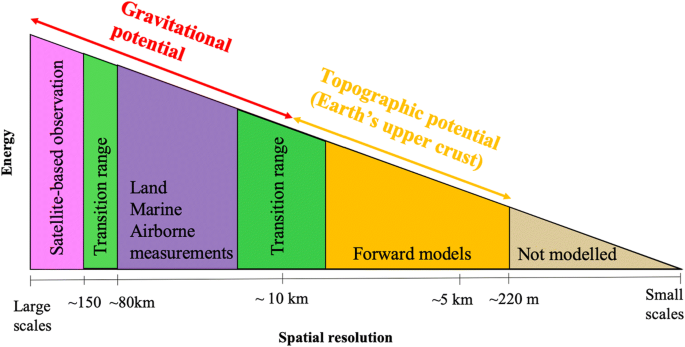Fig. 1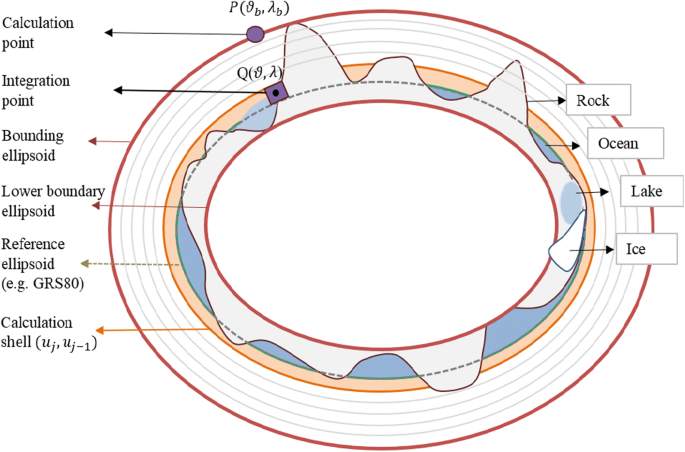Fig. 2

As a rule, existing methods of spectral modelling of the topographic potential in the ellipsoidal approximation are based on series expansions of point positions. Alternating signs in these expansions (e.g., Claessens and Hirt 2013; Wang and Yang 2013) can lead to numerical instabilities. This is why in our method we try to avoid such expansions. Instead, we split the Earth’s interior into a sequence of thin ellipsoidal shells, interpolate the density in each cell of the shell, perform ellipsoidal surface harmonic analysis of these laterally varying density values, and integrate vertically to a surface that is outside of all the masses at the final stage of the processing. We believe that such an approach is free of the above-mentioned numerical issues. By all means, the results are dependent on how well we can compute densities in cells of ellipsoidal shells. However, using the 1 arcmin grids from Earth2014 (Hirt and Rexer 2015), we believe that the density values are good enough to prove the performance of our modelling.

The mass sources used in this paper include the density information below the mean sea level and not only the topography which is traditionally defined as the terrain above the mean sea surface. Therefore, our model in that sense does not represent the gravitational potential of the topography only but includes the bathymetry as well. Accordingly, it is a model that represents the gravitational field of “Earth’s upper crust” partially but not limited to the topography. However, in this paper we will use the commonly used term “topographic gravitational potential”.

In Sect. 2, we present the data used as input to our forward modelling algorithm and continue with the methodology applied in the development of this unique model. In Sect. 3, we compare our high-resolution topographic gravity field potential model, namely ROLI_EllApprox_SphN_3660 (Abrykosov et al. 2019), in spectral and spatial domains with other topographic gravity potential models of similar kind, dV_ELL_Earth2014 (Rexer et al. 2016) and RWI_TOPO_2015 (Grombein et al. 2016) and we explain the common characteristics and differences among these models. In Sect. 4, we present our high-resolution test models that are augmented to higher degree and order using the recent satellite-only GOCE model GO_CONS_GCF_2_DIR_R6 (Förste et al. 2019), the high-resolution combined model EIGEN-6C4, and ROLI_EllApprox_SphN_3660 coefficients. We compare these test models with some of the latest combined high-resolution static gravity field models EIGEN-6C4 and EGM2008 and the “gravito-topo” gravity field model SatGravRET2014 (Hirt et al. 2016b). Section 5 covers the evaluation of the test models with respect to various external datasets, such as GNSS/levelling-derived geoid undulations, terrestrial gravity and deflections of vertical measurements on the Earth surface as well as very high-resolution gravity field functionals of the GGMplus model (Hirt et al. 2013). In Sect. 6, we conclude the paper and give an insight into the upcoming high-resolution combined gravity field model from GFZ that is augmented by forward modelled gravity.

## Data and Methodology

### Data

The mass-source information used in this study is provided by the Earth2014 relief model (Hirt and Rexer 2015). It is a state-of-the-art model of 1 arcmin resolution grids of Earth’s relief in different representations (topography, bedrock, ice) and composed of the most recent DTMs. The Earth2014 is the successor of the Earth2012 and developed at Curtin University, Australia. The model is based on Bedmap2 (Fretwell et al. 2013) bedrock and ice data over Antarctica, GBT v3 Greenland bedrock topography data over Greenland (Bamber et al. 2013), SRTM30_PLUS V9 (Becker et al. 2009) bathymetry over the oceans and major inland lakes, as well as overland in high northern latitudes (except for Greenland), and SRTM V4.1 Topography (Jarvis et al. 2008) over continents/islands. The model provides: (1) elevation grids, (2) Earth shape grids, and (3) ultra-high degree spherical harmonic coefficients up to degree 10,800.

In this study the global grid files are used to forward model the high-frequency gravity field components, which are assumed to be due to the topography and bathymetry. The Earth2014 is freely available in the form of 1’ x 1’ global grids for five files that include SUR (Earth’s surface), BED (Earth’s bedrock), TBI (Topography, bedrock, and ice), ICE (Major ice sheets), and Landtypes mask (MSK2014_landtypes.1min.geod.bin). It is worth noting that there are other density models (Gladkikh and Tenzer 2011; Sheng et al. 2019) which can be used in the modelling as well. In this study, Earth2014 (Hirt and Rexer 2015) is used and the grid files can be downloaded from the Curtin University’s website http://ddfe.curtin.edu.au/gravitymodels/Earth2014/data_1min/.

### Modelling the Potential

Before giving details of the modelling, for the sake of clarity, it is worth noting the difference of the topographic gravity field potential from the gravity field potential. The gravitational potential is generated by all the masses within the Earth’s body following Newton’s Law of Gravitation (Heiskanen and Moritz 1967; Hofmann-Wellenhof and Moritz 2005). Gravity acceleration can be measured directly using dedicated gravimeters at any point and height. Moreover, via satellite-derived observations and different terrestrial techniques (e.g., GNSS/levelling, deflections of vertical), gravity can be indirectly measured or calculated at or above the Earth’s surface. On the contrary, topographic potential (or the potential of Earth’s upper crust) is generated by the masses that are near the Earth’s surface and does not consider the interior potential below the defined reference surface (or the lowermost boundary surface in this study). The geometry and the mass-density relation of those masses for land, oceans and lakes, and ice sheets need to be known reasonably well to arrange the mass sources and apply the mass layer concept forward modelling.

Rexer (2017) summarizes the different approaches used in the development of topographic gravity field potential models. The calculations of such models in spectral and spatial domains can be realized via spherical or ellipsoidal approximations. In the literature, the spherically approximated topographical models have been calculated using different approaches, such as isostatic correction or single-layer and multi-layer approaches, whereas the ellipsoidal approximated topographic models have been computed using the single-layer approach. Rexer (2017) introduced a multi-layer approach using ellipsoidal approximation and generated an ellipsoidal topographic model which is fundamentally in good agreement with our approach presented in this paper. Rexer (2017) uses the same input data to his model that introduces four different density layers and computes height-density functions for different layers, whereas we subdivide the topography and bathymetry into 5 m thick shells and compute the potential of each shell on the surface of the bounding ellipsoid that is located outside of all the masses.

In this contribution, we formulate a new ellipsoidal approximation approach in spectral domain to compute the Earth’s topographic potential that is implied by masses of four layers, namely rock, ocean, lake, and ice sheets using Earth2014 (Fig. 2). As a first step, starting from the deepest point known, 5 m interval shells bounded by 2 confocal ellipsoidal surfaces are located based on the Earth2014 elevation information. The shells cover the Earth’s upper crust from the Mariana trench (− 10,890 m w.r.t. the reference ellipsoid) to approximately the highest peak of the Everest mountain (+ 8250 m w.r.t. the reference ellipsoid). Every single ellipsoid is confocal w.r.t. the reference ellipsoid (GRS80), which is essential in this methodology.

The computation starts with the calculation of the harmonic coefficients of the density of each thin shell bounded by the confocal ellipsoids, and the procedure is repeated for each shell. Wang and Yang (2013) introduced a similar methodology using a constant density with the assumption that the density changes in lateral direction only. In the next step, the ellipsoidal harmonic coefficients of the potential are derived on the Earth’s surface from the harmonic coefficients of the density after the vertical numerical integration of each shell. The potential is expanded for 3825 shells in total on the bounding ellipsoid separately and summed up at the end of the procedure to represent the complete topographic gravity field. As depicted between the bounding and lowermost boundary ellipsoids in Fig. 2, the topography here represents not only the elevation above the mean sea level but also the bathymetry information.

It is worth noting that the spherical harmonic expansion can be considered as a special case of the ellipsoidal harmonic expansion when the semi-minor and semi-major axes of the reference ellipsoid are identical (Heiskanen and Moritz 1967; Claessens 2016). In the final step, the ellipsoidal harmonic coefficients are transformed into spherical harmonic coefficients as described in Jekeli (1988).

The discrepancies in the modelling approaches w.r.t. the above-mentioned references are eventually expected to cause some differences among the models. To be consistent with the commonly used software and the gravity field as well as other topographic models published in International Association of Geodesy (IAG)’s International Centre for Global Earth Models (ICGEM) Service (Ince et al. 2019), we add the potential of the reference ellipsoid (GRS80) to the topographic gravity field. Two models, ROLI_EllApprox_SphN_3660 (topographic model) and ROLI_EllApprox_SphN_3660_plusGRS80 (topographic model together with the normal potential), are available on ICGEM at http://icgem.gfz-potsdam.de/tom_reltopo, and they can also be downloaded from https://doi.org/10.5880/ICGEM.2019.011.

### ROLI Forward Modelling

Rock, Ocean, Lake, and Ice (ROLI) forward modelling is detailed in this section. Let us start with Newton’s integral which expresses the gravity potential at point b as follows:

$$V(b,\vartheta_{b},\lambda_{b}) = G\iiint {\frac{\rho (u,\vartheta,\lambda)}{{\ell (b,\vartheta_{b},\lambda_{b},u,\vartheta,\lambda)}}{\text{d}}v},$$
(1)

where G is the universal gravitational constant. The triplet u, ϑ, λ represents oblate spheroidal coordinates which are commonly called “ellipsoidal coordinates” where u is the semi-minor axis of the ellipsoid, ϑ is the reduced co-latitude, and λ is the longitude of the integration (evaluation) point. The triplet b, ϑb, λb refers to the coordinates of the computation point defined on the bounding ellipsoid that is located outside of all the masses, ℓ is the reciprocal distance, and finally ρ is the density of the volume element defined by (Heiskanen and Moritz 1967; Wang and Yang 2013),

$${\text{d}}v = \left( {u^{2} + E^{2} \cos^{2} \vartheta } \right)\sin \vartheta {\text{d}}\vartheta {\text{d}}\lambda {\text{d}}u,$$
(2)

where E is linear eccentricity (Jekeli 1981).

The definitions given above can be applied for the calculation of the potential of the gravitational field of the Earth. Based on the boundaries defined, the same methodology can be applied to compute the gravitational potential of the topographic or upper crust masses.

Let us now consider that the Earth’s topography is subdivided into shells where the bounding surfaces are confocal ellipsoids w.r.t. the reference ellipsoid and the internal potential that is inside the lowermost boundary ellipsoid is not considered in the model. Due to the reasons mentioned in the literature (Rexer et al. 2016), in this study we approximate the topographic potential field using a reference ellipsoid, known as the ellipsoidal topographic potential (ETP). Accordingly, we represent the field in terms of ellipsoidal harmonic coefficients first and then transform into the spherical harmonic coefficients.

In order to derive the transformation between the two series, we use their alternative representations by means of density integrals (Jekeli 1988). Representation of ellipsoidal harmonic coefficients in terms of density integrals is simply possible once the series expansion of the reciprocal distance is found. Therefore, the ellipsoidal expansion of reciprocal distance defined in Hobson (1931) is one of the key elements for our computations and given as:

\begin{aligned} \frac{1}{\ell } & = \frac{i}{E}\sum\limits_{n = 0}^{\infty } {\sum\limits_{m = 0}^{n} {( - 1)^{m} (2 - \delta_{0m} )(2n + 1)\left[ {\frac{(n - m)!}{(n + m)!}} \right]^{2} Q_{nm} (\mu_{b} )P_{nm} (\mu )} } \\ & \times \left[ {R_{nm} (\vartheta_{b} ,\lambda_{b} )R_{nm} (\vartheta ,\lambda ) + S_{nm} (\vartheta_{b} ,\lambda_{b} )S_{nm} (\vartheta ,\lambda )} \right], \\ \end{aligned}
(3)

where elementary surface harmonics for degree n and order m are defined as

$$\left. {\begin{array}{*{20}c} {R_{nm} (\vartheta ,\lambda )} \\ {S_{nm} (\vartheta ,\lambda )} \\ \end{array} } \right\} = P_{nm} (\cos \vartheta )\left\{ {\begin{array}{*{20}c} {\cos m\lambda } \\ {\sin m\lambda } \\ \end{array} } \right.,$$
(4)

and δ0m is the Kronecker delta. The $$Q_{nm} (\mu_{b} )$$ and $$P_{nm} (\mu )$$ are both functions of imaginary arguments and represent the Legendre function of the second and first kind, respectively, where $$\mu_{b} = i\frac{b}{E}$$ and $$\mu = i\frac{u}{E}$$. Equation (3) is considered in our computation only for the cases b > u where b is constant and refers to the semi-minor axis of the bounding ellipsoid and u represents the semi-minor axis of the ellipsoid of the integration point that changes from layer to layer.

Note that $$\sigma_{b} = \frac{b}{E}$$ and $$\sigma = \frac{u}{E}$$, accordingly $$\mu_{b} = i\sigma_{b} ,\mu = i\sigma$$. The volume element given in Eq. (2) then can be rewritten:

$${\text{d}}v = E^{3} \left( {\sigma^{2} + \cos^{2} \vartheta } \right)\sin \vartheta {\text{d}}\vartheta {\text{d}}\lambda {\text{d}}\sigma .$$
(5)

To simplify the calculation of Eq. (3), let us consider the fully normalized surface harmonics:

\begin{aligned} \left. {\begin{array}{*{20}c} {\bar{R}_{nm} (\vartheta ,\lambda )} \\ {\bar{S}_{nm} (\vartheta ,\lambda )} \\ \end{array} } \right\} & = \bar{P}_{nm} (\cos \vartheta )\left\{ {\begin{array}{*{20}c} {\cos m\lambda } \\ {\sin m\lambda } \\ \end{array} } \right. \\ & = \sqrt {(2 - \delta_{0m} )(2n + 1)\frac{(n - m)!}{(n + m)!}} P_{nm} (\cos \vartheta )\left\{ {\begin{array}{*{20}c} {\cos m\lambda } \\ {\sin m\lambda } \\ \end{array} } \right., \\ \end{aligned}
(6)

which help to formulate the reciprocal distance into:

\begin{aligned} \frac{1}{\ell } & = \frac{i}{E}\sum\limits_{n = 0}^{\infty } {\sum\limits_{m = 0}^{n} {( - 1)^{m} \frac{(n - m)!}{(n + m)!}Q_{nm} (\mu_{b} )P_{nm} (\mu )} } \\ & \left[ {\bar{R}_{nm} (\vartheta_{b} ,\lambda_{b} )\bar{R}_{nm} (\vartheta ,\lambda ) + \bar{S}_{nm} (\vartheta_{b} ,\lambda_{b} )\bar{S}_{nm} (\vartheta ,\lambda )} \right]. \\ \end{aligned}
(7)

As given by Hobson (1931):

\begin{aligned} Q_{nm} (\mu_{b} ) & = ( - 1)^{m} \frac{{2^{n} n!(n + m)!}}{(2n + 1)!}\left( {\mu_{b}^{2} - 1} \right)^{m/2} \\ & \frac{1}{{\mu_{b}^{n + m + 1} }}F\left( {\frac{n + m + 2}{2},\frac{n + m + 1}{2};n + \frac{3}{2};\frac{1}{{\mu_{b}^{2} }}} \right), \\ \end{aligned}
(8)

and

$$P_{nm} (\mu ) = \frac{(2n)!}{{2^{n} n!(n - m)!}}\left( {\mu^{2} - 1} \right)^{m/2} \mu^{n - m} f_{nm} (\mu ).$$
(9)

Substituting μb and μ into Eqs. (8) and (9), these Legendre functions can be rewritten:

$$Q_{nm} (\mu_{b} ) = ( - 1)^{m} \frac{{2^{n} n!(n + m)!}}{(2n + 1)!}\frac{1}{{i^{n + 1} }}q_{nm} (\sigma_{b} ),$$
(10)

and

$$P_{nm} (\mu ) = \frac{(2n)!}{{2^{n} n!(n - m)!}}i^{n} p_{nm} (\sigma ).$$
(11)

To avoid imaginary operations, the reciprocal distance can also be written using real functions qnm and pnm:

\begin{aligned} \frac{1}{\ell } & = \frac{1}{E}\sum\limits_{n = 0}^{\infty } {\sum\limits_{m = 0}^{n} {\frac{1}{2n + 1}q_{nm} (\sigma_{b} )p_{nm} (\sigma )} } \\ & \quad \left[ {\bar{R}_{nm} (\vartheta_{b} ,\lambda_{b} )\bar{R}_{nm} (\vartheta ,\lambda ) + \bar{S}_{nm} (\vartheta_{b} ,\lambda_{b} )\bar{S}_{nm} (\vartheta ,\lambda )} \right], \\ \end{aligned}
(12)

where

$$q_{nm} (\sigma_{b} ) = \frac{{\left( {\sigma_{b}^{2} + 1} \right)^{m/2} }}{{\sigma_{b}^{n + m + 1} }}F\left( {\frac{n + m + 2}{2},\frac{n + m + 1}{2};n + \frac{3}{2}; - \frac{1}{{\sigma_{b}^{2} }}} \right),$$
(13)

and

$$p_{nm} (\sigma ) = (\sigma^{2} + 1)^{m/2} \sigma^{n - m} f_{nm} (\sigma ).$$
(14)

The two real functions $$p_{nm} (\sigma )$$ and $$q_{nm} (\sigma_{b} )$$ represent the radial components of the ellipsoidal expansion and therefore are called “radial functions” in Wang and Yang (2013).

The F in Eq. (13) is the hypergeometric function defined as:

$$F\left( {\frac{n + m + 2}{2},\frac{n + m + 1}{2};n + \frac{3}{2}; - \frac{1}{{\sigma_{b}^{2} }}} \right) = \sum\limits_{l = 0}^{\infty } {\frac{{\left( {\frac{n + m + 2}{2}} \right)_{l} \left( {\frac{n + m + 1}{2}} \right)_{l} }}{{\left( {n + \frac{3}{2}} \right)_{l} l!}}\left( { - \frac{1}{{\sigma_{b}^{2} }}} \right)^{l} } ,$$
(15)

and $$f_{nm} (\sigma )$$ is the finite sum:

$$f_{nm} (\sigma ) = \sum\limits_{l = 0}^{{\left[ {\frac{n - m}{2}} \right]}} {\frac{n!}{l!(n - l)!}\frac{(2n - 2l)!}{(2n)!}\frac{(n - m)!}{(n - m - 2l)!}\frac{1}{{\sigma^{2l} }}} .$$
(16)

Note that in Eq. (15) the alternating sign of the last term of even and odd orders of l can cause instability; therefore, it requires another transformation detailed in the Appendix to adopt the following:

\begin{aligned} & F\left( {\frac{n + m + 2}{2},\frac{n + m + 1}{2};n + \frac{3}{2}; - \frac{1}{{\sigma_{b}^{2} }}} \right) \\ & \quad = \left( {\sqrt {\frac{{\sigma_{b}^{2} }}{{\sigma_{b}^{2} + 1}}} } \right)^{n + m + 1} F\left( {\frac{n + m + 1}{2},\frac{n - m - 1}{2};n + \frac{3}{2};\frac{1}{{1 + \sigma_{b}^{2} }}} \right). \\ \end{aligned}
(17)

Inserting the last expression for the reciprocal distance Eq. (12) into Newton’s integral, the gravitational potential of the topographic masses can finally be expressed in terms of ellipsoidal harmonics:

$$V = {\text{GE}}^{3} \sum\limits_{n = 0}^{N} {\sum\limits_{m = 0}^{n} {\left[ {\bar{A}_{nm} \bar{R}_{nm} (\vartheta_{b} ,\lambda_{b} ) + \bar{B}_{nm} \bar{S}_{nm} (\vartheta_{b} ,\lambda_{b} )} \right]} } ,$$
(18)

where the coefficients are volume integrals:

$$\bar{A}_{nm} = \frac{{q_{nm} (\sigma_{b} )}}{2n + 1}\iiint {\rho (\sigma ,\vartheta ,\lambda )p_{nm} (\sigma )\bar{R}_{nm} (\vartheta ,\lambda )\left( {\sigma^{2} + \cos^{2} \vartheta } \right)\sin \vartheta {\text{d}}\vartheta {\text{d}}\lambda {\text{d}}\sigma },$$
(19)

and

$$\bar{B}_{nm} = \frac{{q_{nm} (\sigma_{b} )}}{2n + 1}\iiint {\rho (\sigma ,\vartheta ,\lambda )p_{nm} (\sigma )\bar{S}_{nm} (\vartheta ,\lambda )\left( {\sigma^{2} + \cos^{2} \vartheta } \right)\sin \vartheta {\text{d}}\vartheta {\text{d}}\lambda {\text{d}}\sigma }.$$
(20)

We compute these coefficients by integrating lateral density variations within a sequence of thin volumetric shells bounded by confocal ellipsoids with semi-minor axes $$\left( {u_{j - 1} ;u_{j} } \right)$$, for lower and upper bounds of the shells. Within each shell, the density is assumed to be independent of u but changes only in the lateral direction. However, from cell to cell of a corresponding grid it can vary. Under these conditions, we can expand $$\rho_{j - 1,j}$$ into series of surface harmonics:

$$\rho_{j - 1,j} (\vartheta ,\lambda ) = \sum\limits_{n = 0}^{N} {\sum\limits_{m = 0}^{n} {\left[ {\bar{\alpha }_{nm}^{j - 1,j} \bar{R}_{nm} (\vartheta ,\lambda ) + \bar{\beta }_{nm}^{j - 1,j} \bar{S}_{nm} (\vartheta ,\lambda )} \right]} } .$$
(21)

Inserting Eq. (21) into Eqs. (19) and (20) and performing the integration over ϑ, λ, the ellipsoidal harmonic coefficients of the potential of the shell $$\left( {u_{j - 1} ;u_{j} } \right)$$ can be represented as:

\begin{aligned} \bar{A}_{nm}^{j - 1,j} & = \frac{{q_{nm} (\sigma_{b} )}}{2n + 1}\left\{ {\bar{\alpha }_{nm}^{j - 1,j} \int\limits_{{\sigma_{j - 1} }}^{{\sigma_{j} }} {\sigma^{2} p_{nm} (\sigma ){\text{d}}\sigma } } \right. \\ & \quad \left. { + \left( {F_{nm}^{ - 2} \bar{\alpha }_{n - 2,m}^{j - 1,j} + F_{nm}^{0} \bar{\alpha }_{nm}^{j - 1,j} + F_{nm}^{ + 2} \bar{\alpha }_{n + 2,m}^{j - 1,j} } \right)\int\limits_{{\sigma_{j - 1} }}^{{\sigma_{j} }} {p_{nm} (\sigma ){\text{d}}\sigma } } \right\}, \\ \end{aligned}
(22)

and

\begin{aligned} \bar{B}_{nm}^{j - 1,j} & = \frac{{q_{nm} (\sigma_{b} )}}{2n + 1}\left\{ {\bar{\beta }_{nm}^{j - 1,j} \int\limits_{{\sigma_{j - 1} }}^{{\sigma_{j} }} {\sigma^{2} p_{nm} (\sigma ){\text{d}}\sigma } } \right. \\ & \quad \left. { + \left( {F_{nm}^{ - 2} \bar{\beta }_{n - 2,m}^{j - 1,j} + F_{nm}^{0} \bar{\beta }_{nm}^{j - 1,j} + F_{nm}^{ + 2} \bar{\beta }_{n + 2,m}^{j - 1,j} } \right)\int\limits_{{\sigma_{j - 1} }}^{{\sigma_{j} }} {p_{nm} (\sigma ){\text{d}}\sigma } } \right\}. \\ \end{aligned}
(23)

Here, factors $$F_{nm}^{ * }$$ have been derived by Claessens (2005):

$$\left. {\begin{array}{*{20}l} {F_{nm}^{ - 2} = \sqrt {\frac{{\left[ {(n - 1)^{2} - m^{2} } \right]\left[ {n^{2} - m^{2} } \right]}}{{(2n - 3)(2n - 1)^{2} (2n + 1)}}} } \hfill \\ {F_{nm}^{0} = \frac{{2n(n + 1) - 2m^{2} - 1}}{(2n - 1)(2n + 3)}} \hfill \\ {F_{nm}^{ + 2} = \sqrt {\frac{{\left[ {(n + 1)^{2} - m^{2} } \right]\left[ {(n + 2)^{2} - m^{2} } \right]}}{{(2n + 1)(2n + 3)^{2} (2n + 5)}}} } \hfill \\ \end{array} } \right\}.$$
(24)

Now, the ellipsoidal harmonic coefficients of the potential, Eq. (18), are sums of the coefficients of each individual shell:

$$\bar{A}_{nm} = \sum\limits_{j} {\bar{A}_{nm}^{j - 1,j} } \quad {\text{and}}\quad \bar{B}_{nm} = \sum\limits_{j} {\bar{B}_{nm}^{j - 1,j} } .$$
(25)

We have performed these computations to degree and order 10,800, which correspond to 1 arcmin resolution of the grids of Earth2014. Finally, to avoid spectral leakage and aliasing and also for practical reasons, we put all the coefficients above degree n = 3600 to be equal to zero: $$\left\{ {\bar{A}_{nm} = \bar{B}_{nm} = 0} \right\}_{n > 3600}$$ and apply Jekeli’s transformation (Jekeli 1988) to obtain spherical harmonic coefficients $$\left\{ {\bar{C}_{nm} , \, \bar{S}_{nm} } \right\}_{n \le 3660}$$.

It is commonly believed that the infinite series for outer potential are convergent at points outside all masses, whereas they are divergent inside the masses. However, this statement is missing the fact that the convergence of the series cannot be guaranteed at or near the Earth’s surface. Well-established theoretical works suggest that the series may be convergent or divergent at the Earth’s surface (Hirt and Kuhn 2017; Hu and Jekeli 2015; Jekeli 1983; Krarup 1969; Moritz 1978, 1980). It is presented both theoretically and numerically that the spherical harmonic (SH) series of gravitational potential converges outside of the sphere that encompasses all field-generating mass (Hu and Jekeli 2015). Inside this particular sphere centred at the origin of the coordinate system (also called Brillouin sphere, Moritz 1980 or bounding sphere, Jekeli 1983), the SH series may or may not converge.

It is worth mentioning that when the series converges inside the bounding ellipsoid, it would be wrong to conclude that it is a representation for the interior gravitational potential in this region. As stated clearly in Moritz (1978), spherical harmonic series always represent a harmonic function which by definition is a solution of Laplace’s equation, whereas the interior potential satisfies Poisson’s equation. The two would not be compatible in the case of continuous density distribution when computing the interior gravitational potential.

According to Krarup (1969), series convergence (or divergence) is an unstable property at or near the Earth surface. Moreover, Moritz (1978) states that the convergence at or near the Earth’s surface can easily change into divergence by an arbitrary small change of the mass distribution. In practice, we never operate with the infinite series but use truncated series. Still, with the expansion of the series up to very high degrees (e.g., beyond 2190, say 10,800), there is a chance that the series may diverge which has been discussed by Hirt and Kuhn (2017) and Jekeli (1983) among others. The debate on how well we can model the very high frequencies is ongoing. It is discussed in the literature that using ellipsoidal harmonics, which are used in the present study, instead of spherical harmonics can improve the convergence regions of the gravity field series expansion (Hu and Jekeli 2015; Reimond and Baur 2016). Accordingly, divergence may start at a higher degree in our approach than in an approach based on spherical harmonics and in our validation we do not see any evidence of divergence at degree 3660. To assess the divergence behaviour of the series expansion, Newtonian integration which is free from the divergence issue can be used (Hirt and Kuhn 2017). Such comparisons are not the topic of the current contribution but shall be considered to evaluate very high degree topographic models.

In this research, based on the commonly used assumption that the high-frequency gravity field components are mainly caused by the topography, we have used the above-presented approach to generate a topographic potential model named ROLI_EllApprox_SphN_3660. The model is developed based on ellipsoidal approximation (“EllApprox”), and the final model represents the mass contributions from average topographic, seawater, freshwater and glacial densities in terms of spherical harmonic coefficients (“Sph”) expanded up to degree (“N”) 3660.

## Comparison of ROLI_EllApprox_SphN_3660 with Other Topographic Gravity Field Models

As mentioned in the introductory section, there are different methodologies which can be applied in the development of topographic gravity field models. During the last few years, these models published by Claessens and Hirt (2013), Grombein et al. (2014, 2016), and Rexer et al. (2016) have been widely used for different applications especially by the geophysicists and become available on the International Association of Geodesy (IAG)’s International Centre for Global Earth Models (ICGEM) service in the list of topographic gravity field models. In order to evaluate our topographic gravity field model, we did comparisons between ROLI_EllApprox_SphN_3660 and dV_ELL_Earth2014 (Rexer et al. 2016) both developed in spectral domain as well as with RWI_TOPO_2015 (Grombein et al. 2016) which is developed in spatial domain.

The dV_ELL_Earth2014 expanded up to spherical harmonic degree 2190 is developed in the spectral domain based on the multi-layer approach. For comparison purposes, the topographic gravity field is computed based on both spherical and ellipsoidal approximation (Rexer et al. 2016). The results presented in the same document state that the ellipsoidal approximation driven model is more realistic. Since its use is more common, the ellipsoidal approximated model represented in terms of ellipsoidal harmonic coefficients is transformed into the spherical harmonic coefficients and have been made publicly accessible on the ICGEM as dV_ELL_Earth2014. Later, an updated version of this model, dV_ELL_Earth2014_5480, developed based on the exact input data, Earth2014, was also published on the same platform (Rexer et al. 2016) which expands the representation of the topographic potential model in terms of spherical harmonic coefficients up to degree 5480.

On the contrary to the dV_ELL_Earth2014, the RWI_TOPO_2015 model expanded up to spherical harmonic degree 2190 is developed based on tesseroids modelled in spatial domain and it is comparable to the model computed in spectral domain. These two models have been chosen to be included in the evaluation procedure since they both are developed based on the multi-layer approach that uses different density values for rock, ocean, lake, and ice.

In our investigations, we follow commonly used comparison techniques such as difference degree amplitudes in the spectral domain and the distribution of the model output differences in spatial domain in terms of different gravity field functionals. Different methods bring different challenges either computationally or methodologically and as expected the modelling techniques have different pros and cons. The comparisons included in this contribution do not aim to answer which model or technique is superior but test the agreement among the models.

### Comparisons in the Spectral Domain

The three models are expanded up to their highest degrees available that is 3660 for ROLI_EllApprox_SphN_3660 and 2190 for RWI_TOPO_2015 and dV_ELL_Earth2014. In theory the ROLI_EllApprox_SphN_3660 can be expanded up to a higher degree such as 10,800 corresponding to the spatial resolution of its input data Earth 2014. However, for practical reasons the calculations are performed up to degree 3660 which is sufficient to prove the methodology followed in this study.

In Fig. 3a, the signal degree amplitudes of the three models are shown in terms of geoid undulations, whereas Fig. 3b shows the degree difference amplitudes between the ROLI_EllApprox_SphN_3660 and the other two topographic gravity field models. The signal degree amplitudes (square root of power per degree n) are computed based on:

$$\sigma_{n} = \sqrt {\sum\limits_{m = 0}^{n} {C_{nm}^{2} + S_{nm}^{2} } } ,$$
(26)

where Cnm and Snm are the unitless coefficients. The signal degree amplitude in terms of geoid height can be computed by:

$$\sigma_{n} (N) = R\sigma_{n} .$$
(27)

The difference degree amplitudes can be computed via the same formulas using the model coefficient differences.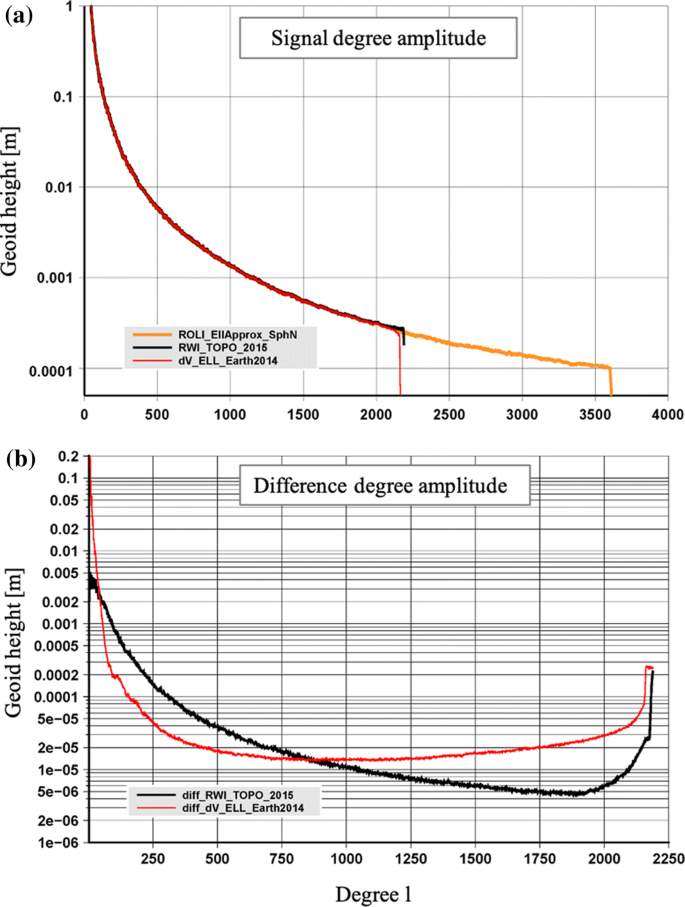Fig. 3

It is worth noting that the scale of the y-axis in Fig. 3b indicates very small amplitude geoid height differences which in principle indicate the good agreement among the models. The agreement between ROLI_EllApprox_SphN_3660 and RWI_Topo_2015 is generally more coherent than with dV_ELL_Earth2014. Notable discrepancies between model differences in the low frequencies are probably due to the modelling techniques and are not the focus of topographic modelling nor this contribution. Fair comparisons should concentrate on the higher degree and order coefficients since the topographic gravity field models do not represent the long-wavelength components of the gravity field with enough accuracy or at all. Therefore, the differences between the ROLI_EllApprox_SphN_3660 and the other two topographic gravity field models in lower degree and order coefficients are not reliable to assess the accuracy of the topographic gravity field model nor the methodology followed in its development. For the higher degree and order coefficients, as shown in Fig. 3b, we observe only sub-mm geoid undulation differences among the three topographic models.

### Comparisons in the Spatial Domain

The differences among the three topographic gravity field models are investigated in the spatial domain in terms of spherically approximated gravity disturbances (Barthelmes 2013). The comparison of gravity disturbances computed from ROLI_EllApprox_SphN_3660 and RWI_TOPO_2015 expanded up to their highest degree and order available is shown in Fig. 4a. In general, the two models are in a very good agreement with each other. The differences are within a reasonable range and related to the high-frequency topographic gravity field contribution located in the mountainous regions. It is worth reminding that ROLI_EllApprox_SphN_3660 model is expanded up to degree 3660, whereas RWI_TOPO_2015 is expanded up to degree 2190 in this comparison. Therefore, ROLI is expected to represent higher-frequency components of the gravity field potential of the terrain. Over the oceans, the differences do not exceed a few mGals nor indicate any particular spatial pattern. They are homogenously distributed.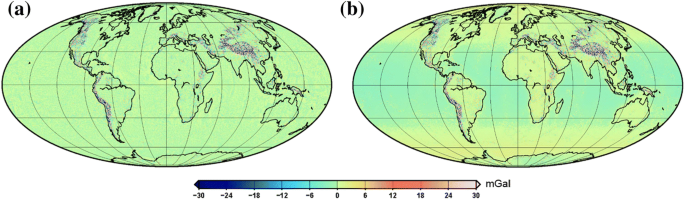Fig. 4

A similar comparison has been performed also with dV_ELL_Earth2014 as shown in Fig. 4b. The differences are within a similar range in the mountainous regions as in the previous comparison shown in Fig. 4a but introduce here a wide band-shaped signature whose origin is unknown to the authors. Similar to the methods applied in the gravity forward modelling literature (Grombein et al. 2016; Rexer et al. 2016), the mass layer concept is realized in this study. Even though there has been extensive research done in the relevant topic, the model developed by Rexer et al. (2016) is the only study that considers different layers (multi-layer aspect) in the ellipsoidal approximation and in the spectral domain which is closest to the methodology followed in this study. Regardless of the band-shaped signature, the two models are in good agreement in the higher-frequency gravity field components.

Rexer et al. (2016) compare the single-layer and multi-layer approaches in the spectral domain in both spherical and ellipsoidal approximations. The single-layer and multi-layer approximations are distinguished by the density values assigned to the mass sources in the modelling. The single-layer approach introduces an average or equivalent density, whereas the multi-layer approach introduces different density values for different mass sources (e.g., rock, ocean). In Earth2014, four different density values, 2670 kg/m3, 1030 kg/m3, 1000 kg/m3, and 917 kg/m3 are assigned to Rock, Ocean, Lake, and Ice Sheet, respectively. Due to the lack of knowledge of Earth’s short-scale density distribution, constant density values for the four layers are used in our modelling with the assumption that the density is height independent and varies only in the lateral direction within a particular shell of interest. It is worth noting that the crustal layer consists of various different types of characteristics and is subject to variations in both lateral and vertical directions. Therefore, the constant density value 2670 kg/m3 is expected to cause the largest approximation errors (Rexer 2017).

## Towards New-Generation High-Resolution Static Gravity Field Models

The above-presented high-resolution topographic gravity field model approximates only the gravity field of the upper crust of the Earth. To approximate the gravity field of the whole Earth body, such topography and density-based forward models need to be combined with gravity data acquired from satellite observations and terrestrial gravity measurements. A simple way for such a combination can be done in the spectral domain by expanding (or augmenting) spherical harmonic coefficient series of traditionally generated satellite-only or combined gravity field models by the coefficients of such kind of novel topographic models. Even in this case, the augmented model would still be an approximation to the gravity field of the whole Earth and would not be the true field. Despite the gravity signal at very short scales not represented by the topographic model would still be missing, the contribution of the topographic model to the combined gravity field model is notable. Hence, the augmentation techniques need to be developed further taking into account higher-resolution density and elevation models.

In this study, using ROLI_EllApprox_SphN_3660 we expand the combined static gravity field model EIGEN-6C4 as well as the latest generation Direct Approach GOCE satellite-only gravity field model GO_CONS_GCF_2_DIR_R6 up to degree and order 3660. The expansion (or augmentation) is not simply done by cutting EIGEN-6C4 or GO_CONS_GCF_2_DIR_R6 at a certain degree and filling-up by the coefficients of the forward model. To avoid any discontinuities, we apply a specific combination technique of the coefficients in a dedicated degree transition range. For instance, in the case of combining a static gravity field with the topographic gravity field model ROLI_EllApprox_SphN_3660, the transition range can be between degrees N1 and N2. In that transition range, the combined spherical harmonic coefficients Cicomb for a specific degree i are calculated according to the following empirical rule:

$$C_{i}^{\text{comb}} = w_{1} C_{i}^{\text{Eigen-6C4}} + w_{2} C_{i}^{\text{ROLI}} ,$$
(28)

where

$$w_{1} = \left( {\frac{{N_{2} - i}}{{N_{2} - N_{1} }}} \right)^{3/2} ,$$
(29)

and

$$w_{2} = 1 - w_{1} .$$

Our two expanded models based on EIGEN-6C4 are labelled EIGEN-6C4.300.500.exp.3660 and EIGEN-6C4.2000.2100.exp.3660 with corresponding transition ranges of N1 = 300 to N2 = 500 and N1 = 2000 to N2 = 2100, respectively. The same kind of combination has been done with GO_CONS_GCF_2_DIR_R6 but for a transition range between degrees 220 and 250. The expanded model based on this satellite-only model is labelled GOCE-DIR6.220.250.exp.3660. The reason to generate different combination examples is to analyse the application of such models for different purposes and regions. For instance, the blended model GOCE-DIR6.220.250.exp.3660 (GOCE_DIR6.exp.3660 in short) and EIGEN-6C4.300.500.exp.3660 are expected to contribute to the gravity field information in Antarctica that is lacking terrestrial gravity measurements.

In our comparisons, we also include the state-of-the-art “gravito-topographic” model SatGravRET2014 (Hirt et al. 2016b) which is developed based on GRACE- and GOCE-derived gravity data and gravitational signals that are retrieved from the 2013 Bedmap2 topography/ice thickness/bedrock model with gravity forward modelling in ellipsoidal approximation. This models’ content is similar to GOCE-DIR6.exp.3660 but the combination is performed in normal equation level using the unconstrained GOCE time-wise model (GO_CONS_GCF_2_TIM_R5, Brockmann et al. 2014). It is worth mentioning that the source global topography model used in GOCE-DIR6.exp.3660 is Earth2014 and it is developed based on Bedmap2 over Antarctica (Hirt et al. 2016b; Klokočník et al. 2018). Due to the contribution of Bedmap2 topography information, we expect clear improvements in the gravity field information over Antarctica w.r.t. EIGEN-6C4 and similarities with SatGravRET2014.

Finally, to evaluate the very high degree and order coefficients of ROLI_EllApprox_SphN_3660, we use the dV_ELL_Earth2014_5480 to augment the EIGEN-6C4 and investigate the differences w.r.t. ROLI_EllApprox_SphN_3660 expanded EIGEN-6C4. The same augmentation procedure mentioned above is applied in the EIGEN-6C4_exp_dV_ELL5480 with a transition range from 2000 to 2100. The list of the models used in the comparisons with their input data is given in Table 1.

### Representation of High-Resolution Augmented Models in Spectral Domain

The longer wavelength components of the augmented gravity fields EIGEN-6C4.300.500.exp.3660 and EIGEN-6C4.2000.2100.exp.3660 are retrieved from EIGEN-6C4 and the short-wavelength components are augmented using the ROLI_EllApprox_SphN_3660. The combinations include a transition range where the two models contribute with a weight scheme applied as presented in Eqs. (28) and (29). The two models mentioned above have transition ranges between the spherical harmonic coefficients 300 and 500, and 2000 and 2100, respectively. Beyond the transition range, the contribution is based solely on the forward model. The same expansion is also valid for the GOCE_DIR6.exp.3660 where the satellite-only model GO_CONS_GCF_2_DIR_R6 and the forward model ROLI_EllApprox_SphN_3660 are combined between degrees 220 and 250. The signal degree amplitude in terms of geoid height is shown for the two expanded models GOCE_DIR6.exp.3660 and EIGEN-6C4.2000.2100.exp.3660 in Fig. 5a, b, respectively, with the transition ranges indicated by the vertical dashed lines.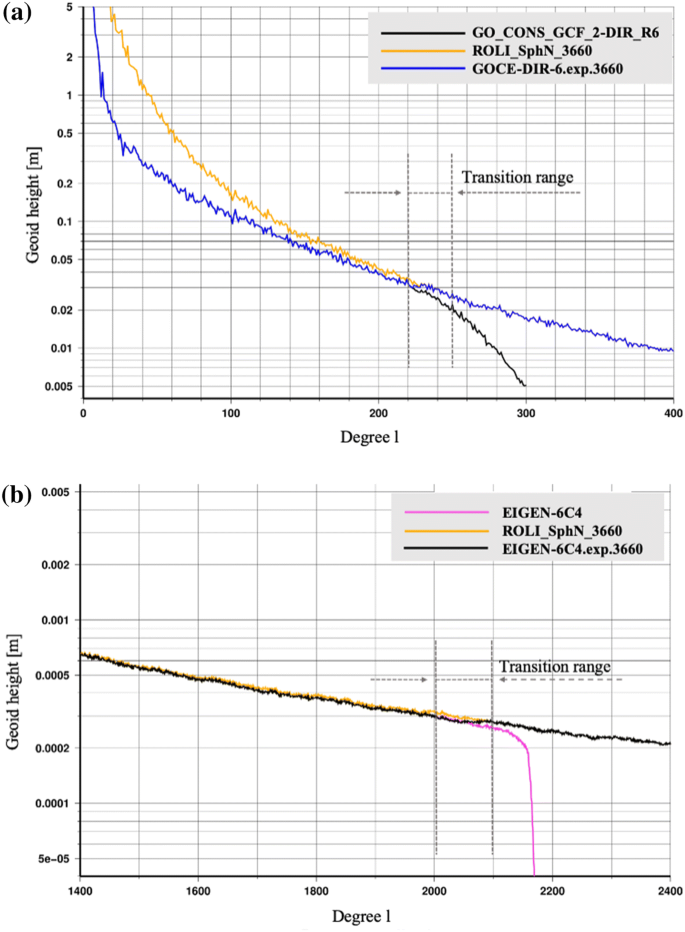Fig. 5

Similar models are tested in the literature and one of the widely used ones, SatGravRET2014 is included in our comparisons. We compare the signal degree amplitudes of EIGEN-6C4, GOCE-DIR6.exp.3660, ROLI_EllApprox_SphN_3660, and SatGravRET2014 in Fig. 6. As mentioned above, topographic gravity field models represent the gravity potential of the topographic mass near the Earth’s surface. Accordingly, long-wavelength components of the gravity field are not well represented in such models as clearly seen in the beginning of the spectrum indicated by the green ellipse in Fig. 6a. The ROLI_EllApprox_SphN_3660 signal represented in orange colour shows a weaker signal than the combined models which indicates the need for the combination. It is also a marker for the possible bandwidth interval where a replacement of long-wavelength components of the topographic model is necessary with other measurements such as satellite-only models which are reliable in this bandwidth interval. Depending on the modelling and its content, different combination intervals need to be tested to retrieve the best approximation for the replacement of the long wavelengths and the degree interval.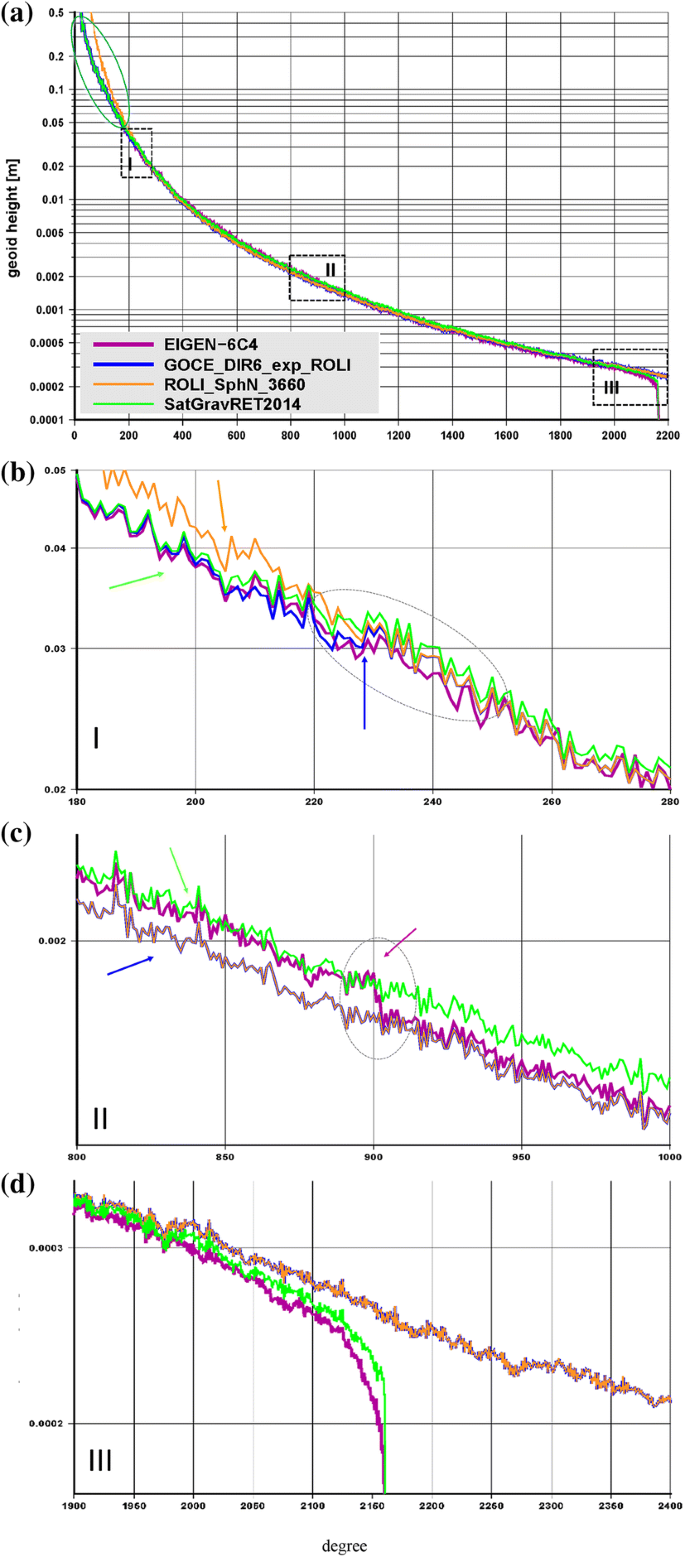Fig. 6

The area I shown in Fig. 6b is the section where the augmentation procedure starts. Up to degree 220, the three models, EIGEN-6C4, GOCE-DIR6.exp.3660, and SatGravRET2014 follow very similar signal characteristics since they all are based on GOCE and GRACE observations in this interval. Starting from the beginning of the transition range of the GOCE-DIR6.exp.3660, the ROLI_EllApprox_SphN_3660 starts contributing to the satellite-only model. The differences are at sub-cm level and indicate the agreement between the combined EIGEN-6C4 and two combined models that are augmented by ROLI_EllApprox_SphN_3660. EIGEN-6C4 starts already benefiting from the contribution of the terrestrial gravity measurements at this transition interval and shows slightly improved signal content up to degree 250.

For the medium-wavelength components shown in the area II, we observe consistency and even slightly better results obtained from the ROLI_EllApprox_SphN_3660 augmented model. As shown in Fig. 6c, GOCE-DIR6.exp.3660 and ROLI_EllApprox_SphN_3660 are overlapped and SatGravRET2014 shows very similar behaviour with a small bias reaching sub-mm level. The source of this bias is unknown to the authors and requires dedicated investigations. The signal content of EIGEN-6C4 indicates a step function at degree around 900 (marked with the magenta arrow). This drop is due to the contribution of EGM2008. It is worth recalling that EIGEN-6C4 is developed based on the contribution from EGM2008 grids. EGM2008 is developed based on satellite data (SLR, GRACE, and altimetry), terrestrial measurements and forward modelled gravity for the areas lacking real gravity measurements. The contribution of the forward model starts at degree 900 and the difference shown in Fig. 6c is due to the differences applied in the forward modelling and the input data included. This step function is already mentioned in the literature (Grombein et al. 2016), and its investigation is beyond the scope of this study.

Finally, in the area III shown in Fig. 6d, we see the signal characteristics of the high-frequency components. The signal degree amplitudes of the high-resolution combined static gravity field models EIGEN-6C4 and SatGravRET2014 start reducing already at about degree 2100, whereas GOCE-DIR6.exp.3660 continues contributing to the high-frequency gravity field information up to ROLI_EllApprox_SphN_3660’s highest degree available.

The signal degree amplitudes of EIGEN-6C4, difference degree amplitudes of GOCE-DIR6.exp.3660 and SatGravRET2014 w.r.t. EIGEN-6C4, and also GOCE-DIR6.exp.3660 and SatGravRET2014 are shown in Fig. 7. The power spectrum of EIGEN-6C4 is given for orientation purpose. In general, the two expanded models based on satellite-only data and forward models are in good agreement and show similar differences w.r.t. the high-resolution combined static gravity field model EIGEN-6C4. The spectral differences are caused by well-known different satellite contributions. As mentioned above, GOCE-DIR6.exp.3660 is based on one of the most recent satellite-only models GO_CONS_GCF_2_DIR_R6 developed via the direct approach which includes the updated GOCE gravity gradients and GFZ’s GRACE-RL06 models (Dahle et al. 2019). On the contrary, SatGravRET2014 is developed based on a previous release GOCE model that is developed using the time-wise approach GO_CONS_GCF_2_TIM_R5 (Brockmann et al. 2014) and GRACE-based static gravity field model ITSG2010 (Mayer-Gürr et al. 2010).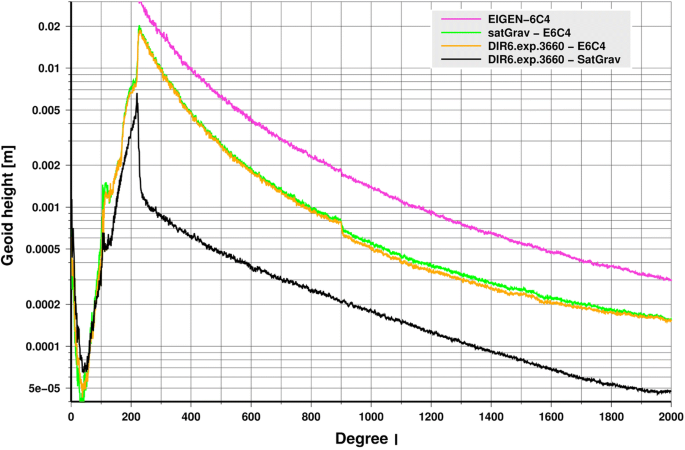Fig. 7

The differences of both forward models (the orange and the green curves) beyond degree 220 w.r.t. EIGEN-6C4 are very close to each other which supports the idea that the forward modelling of both topographic models is generally consistent. This is confirmed by the difference between the two expanded models (the black curve) which is a factor between 5 and 10 times smaller than the differences to EIGEN-6C4.

The spike in all amplitude differences around degree 220 is where the augmentation of the satellite contribution starts by the forward model coefficients. This start degree is approximately the same for both topographic models. However, since the merging approaches are different, we also see a spike in the black curve which is significantly smaller than in the differences w.r.t. EIGEN-6C4. The step around degree 900 in both differences w.r.t. EIGEN-6C4 has been already mentioned before.

To investigate the very high degree and order coefficients of the spectrum provided by ROLI_EllApprox_SphN_3660, we look closer into the spectral differences of the two expanded models that are produced based on EIGEN-6C4 and the two forward models ROLI_EllApprox_SphN_3660 and dV_ELL_Earth2014_5480, respectively. The medium- to high-frequency parts of the degree amplitudes of the two augmented EIGEN-6C4 models and the cumulative differences are shown in Fig. 8 in terms of geoid undulation and gravity anomaly. The augmentation is performed in a similar manner for the two models for the same transition ranges starting from degree 2000 in both cases.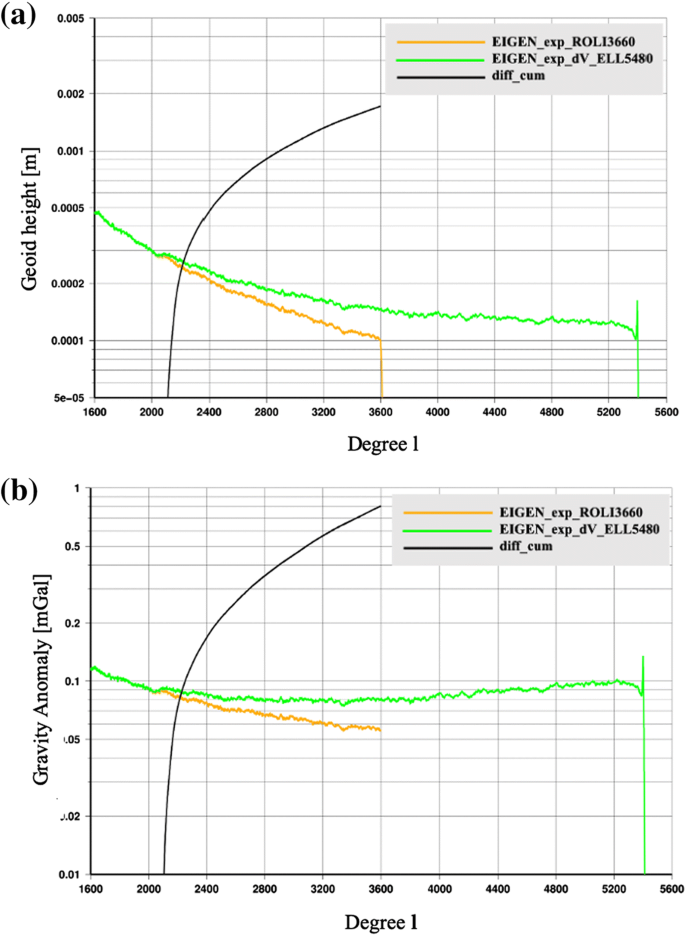Fig. 8

We compute the cumulative spectral differences in terms of geoid height and gravity anomaly that are shown in Fig. 8a, b, respectively. The obtained cumulative differences for geoid undulation and gravity anomaly at degree 3600 are ~ 1.8 cm and 0.8 mGal, respectively. Having in mind that spectral differences are usually interpreted as mean global spatial grid differences of the corresponding functional, we think such numbers are small. The signal degree amplitudes show that the model augmented by ROLI_EllApprox_SphN_3660 indicates a smooth slope-down behaviour at the end of the spectrum at about degree 3600, whereas the model augmented by dV_ELL_Earth2014 indicates a jump at about degree 5400. This is not necessarily a weakness, but rather due to the characteristics of the spectral forward modelling technique at high spectral degree. The impact of this in the spatial domain is to be investigated. What happens if we extend our forward model also to degree/order 5480 will be investigated in a further contribution.

### Representation of the High-Resolution Augmented Models in Spatial Domain

The augmentation of the gravity field models EIGEN-6C4 and GO_CONS_GCF_2_DIR_R6 by the topographic model ROLI_EllApprox_SphN_3660 enhances the spatial resolution of the global representation of the Earth gravity field from approximately 9 km to about 5 km (half-wavelength of the respective maximum spherical harmonic degree). As mentioned above such enhancement is possible based on the assumption that the very high-frequency gravitational field components are due to the topography. The forward model ROLI_EllApprox_SphN_3660_plusGRS80- and the augmented model EIGEN-6C4.2000.2100.ROLI_SphN_3660-derived gravity disturbances computed on the ellipsoid are shown in Fig. 9a and b, respectively. Moreover, the differences between the augmented model and EIGEN-6C4 are shown in Fig. 9c in terms of spherically approximated gravity disturbances. The contribution of the forward model is clearly visible in the high-frequency components of the modelled topographic potential. The mountainous regions indicate differences ranging between − 430 and 320 mGal. Based on the Tscherning–Rapp degree-variance model, mentioned also in Roland (2005) and Torge (1981), the RMS gravity signal strength of the short-scale gravity field within the spatial scales 1–10 km is estimated to be 11.8 mGal. The same estimation for geoid undulation is about 2.6 cm (Hirt et al. 2014).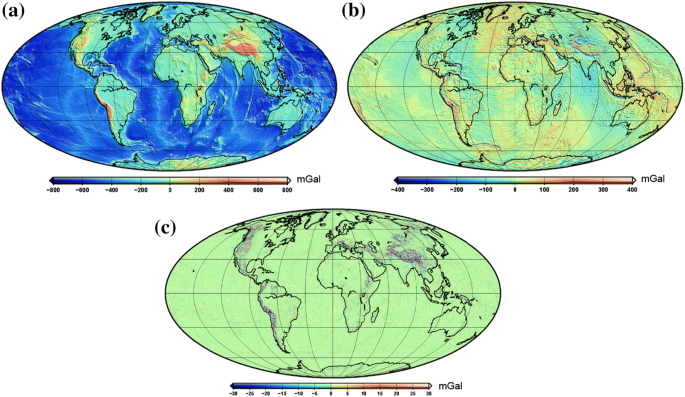Fig. 9

Similar comparisons are performed for Antarctica, which is the most difficult continent to collect data due to its harsh environment especially in the GOCE polar gap regions. In this region, we compared EIGEN-6C4 gravity anomalies with those from GOCE-DIR6.exp.3660 which clearly shows the refinements in the details except for the two regions marked by circles where the bedrock topography data are not based on high-resolution elevation measurements but gravity data (Fretwell et al. 2013). Note that a conceptually similar augmentation has been also presented in Hirt et al. (2016b). Due to the contribution of Bedmap2, higher resolution is reached in the present study.

The recent high-resolution gravity field models like EGM2008 and EIGEN-6C4 do not contain any terrestrial gravity data in Antarctica (Fig. 10). The topographic modelling (or forward modelling) enhances the spatial resolution over the land to a great extent as shown in Fig. 10b. The recently published terrestrial gravity data of the Antarctic Gravity Project (Scheinert et al. 2016) open the possibility to examine the reliability of our topographic model and the method applied in its development in this particular area. Such an evaluation by pointwise comparison of Antarctic gravity anomaly data with the extended model GOCE-DIR6.exp.3660 is provided in Sect. 4.3.3.Fig. 10

Another analysis is performed between the two combined models, EIGEN-6C4.300.500.exp.3660 and EIGEN-6C4.2000.2100.exp.3660. The contributions of the very high-frequency components are eliminated in order to investigate the differences in medium- to high-frequency components of the gravity field where ROLI_EllApprox_SphN_3660 can contribute to new-generation high-resolution combined models. As indicated in Pavlis et al. (2012), the Tian Shan and Pamir areas of northwest China lack terrestrial gravity measurements and they are handled as filled-in areas using forward modelling technique in EGM2008. The EIGEN-6C4 model also includes this filled-in information indirectly by the inclusion of EGM2008 grids.

In this comparison, we present the spherically approximated gravity disturbance differences between the DTM2006.0 filled-in EGM2008 and Earth2014 filled-in EIGEN-6C4.300.500.exp.3660 model. As mentioned in Grombein et al. (2016), the differences between the DTM2006.0 and Earth2014 are due to refined bathymetry depths retrieved from new ship sounding measurements and also due to the improved bedrock information retrieved in the area of Greenland and Antarctica ice masses. The rest of the globe is covered by the SRTM data in both models and the differences are much smaller. However, due to the higher resolution of Earth2014, regions of the highly variable topography are refined. Therefore, in our example, we expect to see the improvement due to the higher-resolution elevation data used in the forward modelling.

The differences presented in Fig. 11 represent the contribution of ROLI_EllApprox_SphN_3660 into EIGEN-6C4 in this area. One can clearly see that the forward modelled ROLI_EllApprox_SphN_3660 complements the information included in EIGEN_6C4 in the medium-wavelength gravity field components and it is recommended to be included in the next-generation high-resolution static gravity field models. It is worth recalling that the contribution comes from the high-resolution elevation data. Considering the existence of very limited gravity measurements in this particular area, filling in will again be part of the next-generation gravity field models, such as EIGEN-X and EGM2020.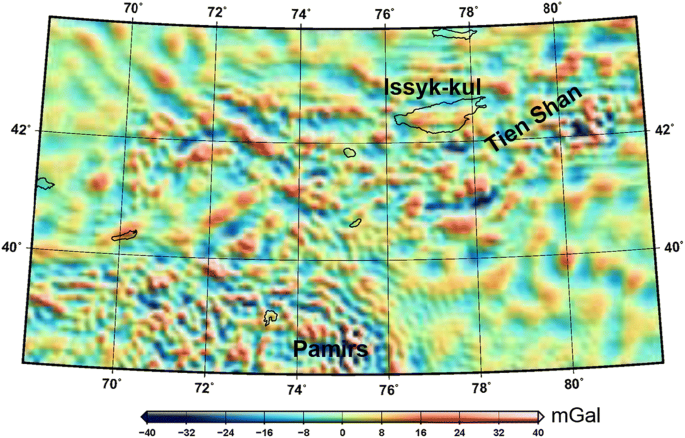Fig. 11

### Evaluation of the Augmented Models w.r.t. Terrestrial Measurements

The expanded models EIGEN-6C4.2000.2100.exp.3660 and GOCE-DIR6.exp.3660 are further evaluated using different sets of terrestrial data. These external data are reliable markers to test the quality of the gravity field models in question, of course within the uncertainty bounds of the external datasets. In our comparisons, EGM2008 and EIGEN-6C4 are also included for reference purposes to highlight the differences/improvements due to the augmentation with our topographic model.

We include three different external terrestrial measurement sets in our comparisons, namely GNSS/levelling-derived geoid undulations, gravity measurements, and deflections of the vertical which are collected over different areas. We also include the very high-resolution gravity field model GGMplus (Hirt et al. 2013) gravity acceleration values in a dedicated mountainous area for comparison purposes. The computations of the respective functionals derived from the spherical harmonic coefficient series were done according to formulae given in Barthelmes (2013).

#### Evaluation w.r.t. Worldwide GNSS/Levelling-Derived Geoid Undulations

Geoid undulations computed from the augmented model as well as from EGM2008 and EIGEN-6C4 are compared with GNSS/levelling-derived geoid undulations (height anomalies for Australia and Germany) collected from different countries. The summary of the RMS values of the residuals is given in Table 2. The results indicate sub-cm level improvement in the agreement of the expanded model EIGEN6C4.2000.2100.exp.3660 computed geoid undulation values than EIGEN-6C4 and EGM2008 when compared w.r.t. GNSS/levelling-derived values in USA, Germany, and Canada. Only minor improvement is expected since the geoid comparison is not the ideal marker to monitor the contribution of the high-frequency gravity field components. As is well known, the geoid is a smooth functional of the gravity field and the assessments need to be extended using other functionals such as gravity anomalies or deflections of the vertical. Moreover, the testing power of the GNSS/levelling geoid undulations is limited due to various other error sources which are not investigated in this contribution. Nevertheless, the consistency between EIGEN-6C4.2000.2100.exp.3660 and EIGEN-6C4 and EGM2008 is a good indicator to verify the methodology followed in the development of ROLI_EllApprox_SphN_3660.

#### Evaluation w.r.t. Gravity Measurements in Switzerland

Because the above comparisons with GNSS/levelling-derived geoid undulations are not satisfactory, comparisons with gravity measurements collected on the Earth’s surface are performed for verifying the agreement between the values computed from our augmented models and the independent measurements. In contrast to geoid undulations, gravity anomalies are more advantageous for the evaluation of high-frequency component gravity field models. For this purpose, we compare our expanded model EIGEN-6C4.2000.2100.exp.3660 with gravity data from Switzerland.

The dataset “swisstopo2004” was provided by courtesy of the Federal Office of Topography, Wabern, Switzerland (Swisstopo). It consists of about 30.000 absolute gravity point records given at the Earth’s surface (status end of 2003). Since the height component of the primarily provided data was given in terms of physical height only, Swisstopo provided ellipsoidal heights on our request as well ( U. Marti, Swisstopo, personal communication, 2017).  For our comparison with the global gravity field models, we create two gravity data point subsets:

• swisstopo2004 subset 1 = 712 data points out of the entire Swiss dataset where the heights were estimated by GPS

• swisstopo2004 subset 2 = The remaining 30,886 data points except those with indicated problems.

The spatial distributions of the two subsets and the differences between the expanded model EIGEN-6C4.2000.2100.exp.3660 and the terrestrial measurements with a colour code are shown in Fig. 12. For both datasets we calculate the differences between the absolute gravity values computed from the expanded model EIGEN-6C4.2000.2100.exp.3660 as well as such from EGM2008 and EIGEN-6C4 and the gravity measurements provided on the benchmarks. As shown in the figure, the residuals are larger in the mountainous areas. The use of higher degree/order expansion of topographic model in future will reduce the discrepancies between the blended model and ground-truth gravity measurements.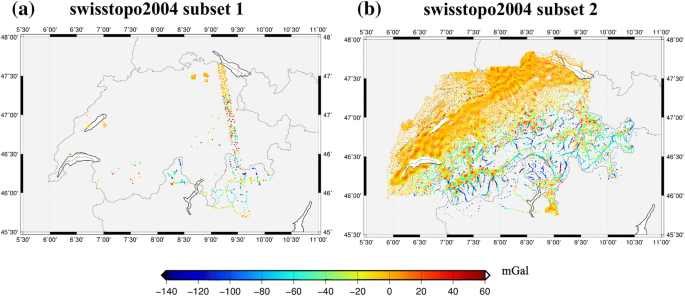Fig. 12

The comparisons in terms of RMS and mean values are given in Table 3. The residuals indicate significant improvement in the augmented model (reduction of RMS and mean values by 20–30%) for both data sets, which clearly proves the valuable contribution of the topographic gravity field modelling.

#### Evaluation w.r.t. the Gravity Measurements in Antarctica

We use the recently published gravity anomaly data for Antarctica (Scheinert et al. 2016) to evaluate the topographic model in this region. Since EIGEN-6C4 does not contain any terrestrial gravity data in this particular area, we conduct the comparison with the expanded model GOCE-DIR6.exp.3660, where the forward model contribution starts already at degree 220. We took the 95,152 gravity anomaly data points out of the continental part of the above-mentioned Antarctic dataset and calculate their differences to the expanded model as well as to EGM2008 and EIGEN-6C4.

Figure 13 shows the spatial distribution of these gravity anomaly differences, whereas the RMS values of the respective gravity anomaly residuals are provided in Table 4. The obtained RMS values are decreased for GOCE-DIR6.exp.3660 w.r.t. EIGEN-6C4 and EGM2008 by about 20–25%, demonstrating that our topographic model improves the representation of the Earth’s gravity field in Antarctica.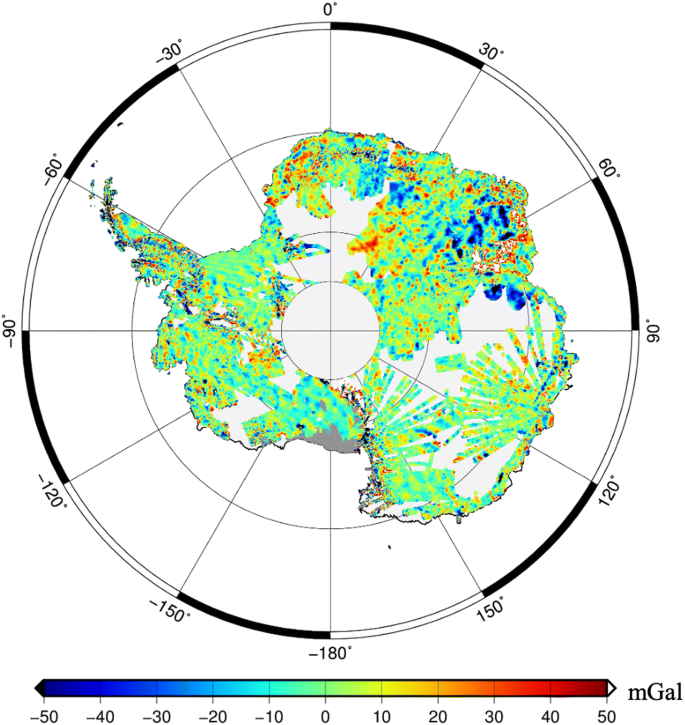Fig. 13

#### Evaluation w.r.t. the Deflections of the Vertical Measurements in Germany

Further evaluations are performed w.r.t. deflections of the vertical measurements provided by Voigt (2013) which are also presented in Voigt and Denker (2014, 2018). As shown in Fig. 14, the measurements cover two profiles along different topographic features in the north–south and east–west directions in Germany that consist of 216 and 154 data points, respectively. The comparisons are summarized in Table 5. The residual RMS values are reduced for the expanded model by about 3–30% for both profiles and both directions. This shows remarkable improvement w.r.t. EGM2008 and EIGEN-6C4 and verifies the enhancement due to the incorporating topographic gravity field potential into high-resolution static gravity field model.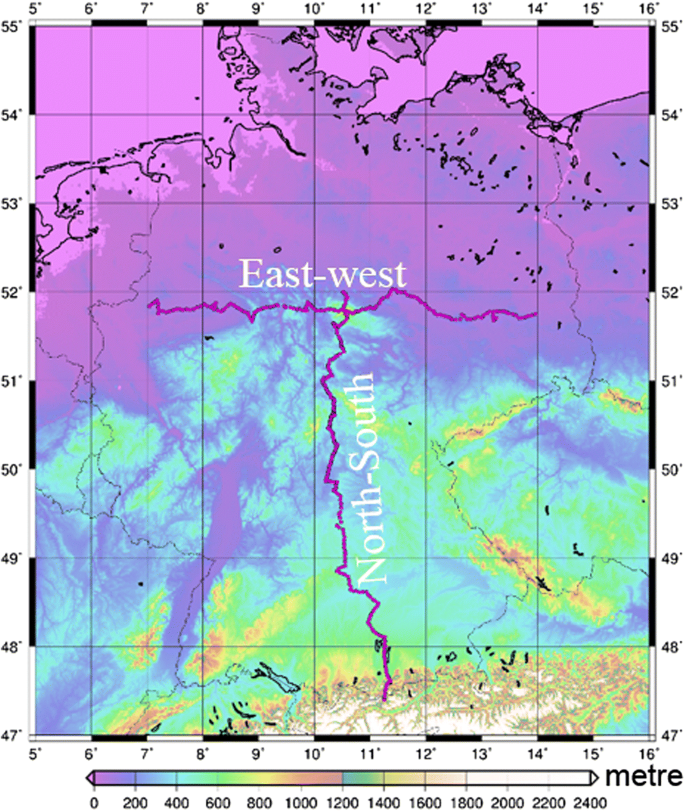Fig. 14

#### W.r.t. the High-Resolution Global Gravity Field Model GGMplus

In this section, we compare our expanded model EIGEN-6C4.2000.2100.exp.3660 with unique GGMplus-derived gravity accelerations. GGMplus includes (a) 7 years of GRACE satellite data (ITG2010s, Mayer-Gürr et al. 2010), (b) 2 years of GOCE satellite data (TIM-R4, Pail et al. 2011), (c) EGM2008 global gravity model (Pavlis et al. 2012), (d) 7.5 arcsec SRTM topography data (V4.1 release, Jarvis et al. 2008), and (e) 30 arcsec SRTM30_PLUS bathymetry data (V7.0 release, Becker et al. 2009) over near-coastal areas. As mentioned in Hirt (2012), GRACE/GOCE and EGM2008 were combined at the Earth’s topography in the spectral band interval between 2 and 2190 using the gradient approach to fifth order (Hirt 2012). High-pass-filtered SRTM topography and SRTM30_PLUS bathymetry are used as data source for forward modelling of short-scale gravity effects using a modified RTM approach (Hirt 2013). These short-scale gravity field components are available as ERTM2160 (Earth Residual Terrain Modelled gravity field) at https://www.bgu.tum.de/iapg/forschung/schwerefeld/ggmplus/. The conversion of high-pass-filtered topography/bathymetry to gravity effects as mentioned in Forsberg (1984) is performed via parallelization and use of advanced computational resources of the iVEC supercomputing facility in Perth, Australia. For further information on the construction of GGMplus, the reader is referred to Hirt et al. (2013).

Our investigations are performed in a particular mountainous region that is also mentioned in Hirt et al. (2013). This area includes the Mount Everest and shows a variety of different characteristics of topography within a relatively small area. GGM plus is a unique ultra-high-resolution gravity field model made available in terms of gridded gravity field functionals in the area between− 60° S and 60° N and resolves the Earth’s gravity field up to ~ 200 m spatial resolution. The grid used in our example is downloaded from Curtin University, http://ddfe.curtin.edu.au/gravitymodels/GGMplus/.

In our comparisons, using cubic interpolation we downsampled the GGMplus grid into our expanded model’s resolution which is about 5 km and compared the two sets of gravity accelerations. The ggm_read.m provided in the GGMplus package is used for this purpose. Figure 15a represents the GGMplus gravity accelerations downsampled to 5 arcmin resolution, whereas Fig. 15b shows the gravity acceleration computed based on the combined model EIGEN-6C4.2000.2100.exp.3660. The differences between the two are presented in Fig. 15c which indicate small peaks in the highest mountainous areas that are probably not represented in our combined model due to the lower resolution. The rest of the residuals are much smaller in the southern and northern areas verifying once again the quality of our topographic model and its potential application for the development of the future high-resolution combined gravity field models. The use of higher-resolution ROLI_EllApprox_SphN (degree 5480) is expected to reduce such differences.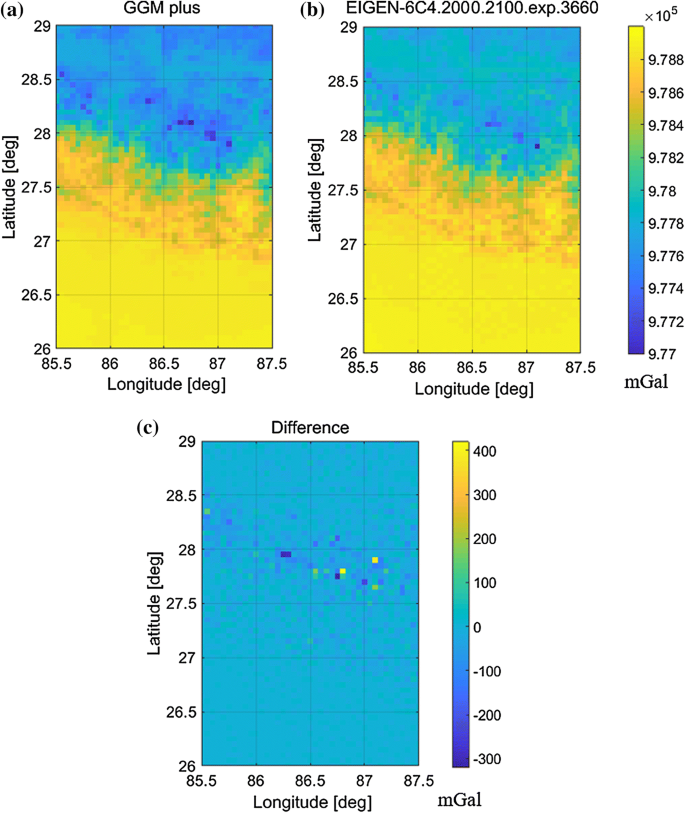Fig. 15

## Conclusions and Outlook

Topographic gravity field models represent the gravitational potential generated by the attraction of the Earth’s topographic masses which may consist of rock, ocean, lake and ice. The gravity is computed based on the shape of the topography (e.g., digital elevation model) and the mass-density knowledge. Assuming that the high-frequency gravity field components are mainly caused by the topography, such models can then be used to complement high-resolution combined static gravity field models for the very high-frequency components of the gravity field as well as to fill in information into regions lacking terrestrial gravity measurements such as Antarctica. The refined features are not limited to the areas lacking gravity measurements, but they enhance the gravity field information in all mountainous regions with varying topography. The reliability of the developed topographic gravity field model is based on the resolution of the input data, the uncertainties of the digital elevation model, validity of the density models, the mass arrangement approach (spherical and ellipsoidal) and the forward modelling technique.

In this study, to compute the topographic gravity effect we presented an alternative approach to the existing ones. We forward modelled the gravity field of Earth's upper crust based on ellipsoidal approximation in spectral domain using multi-layer density information together with globally distributed elevation data. We developed a new algorithm that expresses the gravity field of thin shells in terms of ellipsoidal harmonic coefficients on the Earth’s surface which are then converted into spherical harmonic coefficients expanded up to degree 3660. We think expanding the potential of the Earth’s interior split into a sequence of ~ 5 m thick shells avoids numerical instabilities and avoids the numerical issues existing in other models. We assessed the performance of our forward model ROLI_EllApprox_SphN_3660 with independent datasets and other similar models selected from the literature. The validation results indicate methodological differences among the models but confirm the sub-mm agreement per degree in terms of geoid height. The forward model, ROLI_EllApprox_SphN_3660, is further used to augment the high-resolution static gravity field model EIGEN-6C4 and the satellite-only model GO_CONS_GCF_2_DIR_R6. The external dataset comparisons of the augmented models w.r.t. ground-truth data clearly indicate the improvements coming from the topographic model which confirms their potential use to complement high-resolution static gravity field models. The comparisons w.r.t. GNSS/levelling-derived geoid undulations, terrestrial gravity data, and deflections of the vertical measurements showed improvement in terms of RMS and mean values of up to 30% verifying the forward model contribution in the medium and high-frequency interval that are applicable to small spatial scale features.

We forward modelled the high-frequency components of the gravity field considering mass distributions of average topographic, seawater, freshwater, and glacial densities. Since the model is limited to the information coming from the density values and their variation, further improvement to the current contribution is possible by introducing more realistic topographic density models to our methodology. In future work, we plan to introduce a higher-resolution laterally varying global topographic density model (e.g., UNB_TopoDens) and to forward model the topographic gravity field up to a higher degree using the approach presented in this paper. With this we expect to receive a more realistic topographic gravity field together with uncertainty estimation derived from UNB_TopoDens (Sheng et al. 2019) which then will be used to augment EIGEN-6C4 and further develop the EIGEN-X series. Moreover, the methodology presented in this paper allows us to introduce different density values for different shells positioned at different depths. Such an approach would improve the modelling of the gravitational field of the topography. Our approach can also be used to model the gravitational field of the layers below the topography which is used for geophysical and geodynamic investigations (e.g., Mooney and Kaban 2010).

The models developed in this contribution, ROLI_EllApprox_SphN_3660 and ROLI_EllApprox_SphN_3660_plusGRS80, are available on the ICGEM Service (http://icgem.gfz-potsdam.de/tom_reltopo) and can be accessed and downloaded freely also from http://doi.org/10.5880/ICGEM.2019.011.

## References

1. Abramovitz M, Stegun IA (1964) Handbook of mathematical functions with formulas, graphs and mathematical tables. Tenth Printing, December 1972, with corrections

2. Abrykosov O, Ince ES, Förste C, Flechtner F (2019) ROLI topographic gravity field model, from four-layer Earth decomposition. GFZ Data Serv. https://doi.org/10.5880/ICGEM.2019.011

3. Balmino G, Vales N, Bonvalot S, Briais A (2012) Spherical harmonic modelling to ultra-high degree of Bouguer and isostatic anomalies. J Geodesy 86(7):499–520. https://doi.org/10.1007/s00190-011-0533-4

4. Bamber JL, Griggs JA, Hurkmans RT et al (2013) A new bed elevation dataset for Greenland. The Cryosphere 7:499–510. https://doi.org/10.5194/tc-7-499-2013

5. Barthelmes F (2013) Definition of functionals of the geopotential and their calculation from spherical harmonic models: theory and formulas used by the calculation service of the International Centre for Global Earth Models (ICGEM); http://icgem.gfz-potsdam.de/ICGEM/; revised Edition, (Scientific Technical Report; 09/02), Deutsches GeoForschungsZentrum GFZ, Potsdam, p 32. http://doi.org/10.2312/GFZ.b103-0902-26

6. Becker JJ, Sandwell DT, Smith WHF et al (2009) Global bathymetry and elevation data at 30 arc seconds resolution: SRTM30_PLUS. Marine Geodesy 32(4):355–371. https://doi.org/10.1080/01490410903297766

7. Brockmann JM, Zehentner N, Höck E, Pail R, Loth I, Mayer-Gürr T, Schuh WD (2014) EGM_TIM_RL05: an independent geoid with centimeter accuracy purely based on the GOCE mission. Geophys Res Lett 41(22):8089–8099. https://doi.org/10.1002/2014GL061904

8. Claessens SJ (2005) New relations among associated Legendre functions and spherical harmonics. J Geod 79:398–406. https://doi.org/10.1007/s00190-005-0483-9

9. Claessens SJ (2016) Spherical harmonic analysis of a harmonic function given on a spheroid. Geophys J Int 206(1):142–151. https://doi.org/10.1093/gji/ggw126

10. Claessens SJ, Hirt C (2013) Ellipsoidal topographic potential: new solutions for spectral forward gravity modeling of topography with respect to a reference ellipsoid. J Geophys Res Solid Earth 118(11):5991–6002. https://doi.org/10.1002/2013JB010457

11. Dahle C, Murböck M, Flechtner F, Dobslaw H, Michalak G, Neumayer KH, Abrykosov O, Reinhold A, König R, Sulzbach R, Förste C (2019) The GFZ GRACE RL06 monthly gravity field time series: processing details and quality assessment. Remote Sens 11(18):2116. https://doi.org/10.3390/rs11182116

12. Featherstone WE, Brown NJ, McCubbine JC, Filmer MS (2018) Description and release of Australian gravity field model testing data. Aust J Earth Sci 65(1):1–7. https://doi.org/10.1080/08120099.2018.1412353

13. Fecher T, Pail R, Gruber T (2013) Global gravity field modeling based on GOCE and complementary gravity data. Int J Appl Earth Obs Geoinf 35:120–127. https://doi.org/10.1016/j.jag.2013.10.005

14. Forsberg R (1984) A study of terrain reductions, density anomalies and geophysical inversion methods in gravity field modelling. OSU Report 355. Department of Geodetic Science and Surveying, Ohio State University, Columbus

15. Förste C, Bruinsma S, Abrikosov O, Lemoine JM, Marty J, Flechtner F, Balmino G, Barthelmes F, Biancale R (2014) EIGEN-6C4 the latest combined global gravity field model including GOCE data up to degree and order 2190 of GFZ Potsdam and GRGS Toulouse. GFZ Data Serv. https://doi.org/10.5880/icgem.2015.1

16. Förste C, Abrikosov O, Bruinsma S, Dahle C, König R, Lemoine JM (2019) ESA’s Release 6 GOCE gravity field model by means of the direct approach based on improved filtering of the reprocessed gradients of the entire mission (GO_CONS_GCF_2_DIR_R6). GFZ Data Serv. https://doi.org/10.5880/ICGEM.2019.004

17. Fretwell P, Pritchard HD, Vaughan DG, Bamber JL, Barrand NE, Bell R et al (2013) Bedmap2: improved ice bed, surface and thickness datasets for Antarctica. The Cryosphere 7(1):375–393. https://doi.org/10.5194/tc-7-375-2013

18. Gladkikh V, Tenzer R (2011) A mathematical model of the global ocean saltwater density distribution. Pure Appl Geophys 169(1–2):249–257. https://doi.org/10.1007/s00024-011-0275-5

19. Grombein T, Luo X, Seitz K, Heck B (2014) A wavelet-based assessment of topographic-isostatic reductions for GOCE gravity gradients. Surv Geophys 35(4):959–982. https://doi.org/10.1007/s10712-014-9283-1

20. Grombein T, Seitz K, Heck B (2016) The rock–water–ice topographic gravity field model RWI_TOPO_2015 and its comparison to a conventional rock-equivalent version. Surv Geophys 37(5):937–976. https://doi.org/10.1007/s10712-016-9376-0

21. Grombein T, Seitz K, Heck B (2017) On high-frequency topography-implied gravity signals for a height system unification using GOCE-based global geopotential models. Surv Geophys 38(2):443–477. https://doi.org/10.1007/s10712-016-9400-4

22. Heiskanen WA, Moritz H (1967) Physical geodesy. WH Freeman & Co., San Francisco

23. Hirt C (2012) Efficient and accurate high-degree spherical harmonic synthesis of gravity field functionals at the Earth’s surface using the gradient approach. J Geodesy 86(9):729–744. https://doi.org/10.1007/s00190-012-0550-y

24. Hirt C (2013) RTM gravity forward-modeling using topography/bathymetry data to improve high-degree global geopotential models in the coastal zone. Marine Geodesy 36(2):1–20. https://doi.org/10.1080/01490419.2013.779334

25. Hirt C, Rexer M (2015) Earth 2014 1 arc-min shape, topography, bedrock and ice-sheet models—available as gridded data and degree-10,800 spherical harmonics. Int J Appl Earth Obs Geoinf 39:103–112. https://doi.org/10.1016/j.jag.2015.03.001

26. Hirt C, Kuhn M (2017) Convergence and divergence in spherical harmonic series of the gravitational field generated by high-resolution planetary topography—a case study for the Moon. J Geophys Res Planets 122(8):1727–1746. https://doi.org/10.1002/2017JE005298

27. Hirt C, Gruber T, Featherstone W (2011) Evaluation of the first GOCE static gravity field models using terrestrial gravity, vertical deflections and EGM2008 quasigeoid heights. J Geodesy 85:723–740. https://doi.org/10.1007/s00190-011-0482-y

28. Hirt C, Claessens SJ, Fecher T, Kuhn M, Pail R, Rexer M (2013) New ultra-high resolution picture of 2 Earth’s gravity field. Geophys Res Lett. https://doi.org/10.1002/grl.50838

29. Hirt C, Kuhn M, Claessens S, Pail R, Seitz K, Gruber T (2014) Study of the Earth’s short-scale gravity field using the ERTM2160 gravity model. Comput Geosci 73:71–80. https://doi.org/10.1016/j.cageo.2014.09.001

30. Hirt C, Reußner E, Rexer M, Kuhn M (2016a) Topographic gravity modelling for global Bouguer maps to degree 2,160: validation of spectral and spatial domain forward modelling techniques at the 10 microGal level. J Geophys Res Solid Earth 121:B51763. https://doi.org/10.1002/2016JB013249

31. Hirt C, Rexer M, Scheinert M, Pail R, Claessens S, Holmes S (2016b) A new degree-2190 (10 km resolution) gravity field model for Antarctica developed from GRACE, GOCE and Bedmap2 data. J Geodesy 90(2):105–127. https://doi.org/10.1007/s00190-015-0857-6

32. Hirt C, Rexer M, Claessens S, Rummel R (2017) The relation between degree-2160 spectral models of Earth’s gravitational and topographic potential: a guide on global correlation measures and their dependency on approximation effects. J Geodesy 91(10):1179–1205. https://doi.org/10.1007/s00190-017-1016-z

33. Hobson EW (1931) The theory of spherical and ellipsoidal harmonics. Cambridge University Press, Cambridge

34. Hofmann-Wellenhof B, Moritz H (2005) Physical geodesy. Springer, New York; ISBN-10 3-211-23584-1 resp. ISBN-13 978-3-211-23584-3

35. Hu X, Jekeli C (2015) A numerical comparison of spherical, spheroidal and ellipsoidal harmonic gravitational field models for small non-spherical bodies: examples for the Martian moons. J Geodesy 89(2):159–177. https://doi.org/10.1007/s00190-014-0769-x

36. Ihde J, Adam J, Gurtner W, Harsson BG, Sacher M, Schlüter W,Wöppelmann G (2002) The height solution of the European Vertical Reference Network (EUVN). Mitteilungen des BKG, Bd. 25, EUREF Publication No. 11/I, Frankfurt a. M., pp 53–79

37. Ince ES, Barthelmes F, Reißland S, Elger K, Förste C, Flechtner F, Schuh H (2019) ICGEM—15 years of successful collection and distribution of global gravitational models, associated services, and future plans. Earth Syst Sci Data 11:647–674. https://doi.org/10.5194/essd-11-647-2019

38. Jarvis A, Reuter HI, Nelson A, Guevara E (2008) Hole-filled SRTM for the globe v4.1. Available from the CGIAR‐SXI SRTM 90 m database at http://srtm.csi.cgiar.org

39. Jekeli C (1981) The downward continuation to the Earth’s surface of truncated spherical and ellipsoidal harmonic series of the gravity and height anomalies. Ohio State Univ Report 323

40. Jekeli C (1983) A numerical study of the divergence of spherical harmonic series of the gravity and height anomalies at the Earth’s surface. Bull géodésique 57(1–4):10–28

41. Jekeli C (1988) The exact transformation between ellipsoidal and spherical harmonic expansions. Manuscr Geod 13:106–113

42. Klokočník J, Kostelecký J, Cilek V, Bezděk A, Pešek I (2018) Gravito-topographic signal of the Lake Vostok area, Antarctica, with the most recent data. Polar Sci 17:59–74. https://doi.org/10.1016/j.polar.2018.05.002

43. Krarup T (1969) A contribution to the mathematical foundation of physical geodesy. Springer, Berlin

44. Martinec Z, Grafarend EW (1997) Solution to the Stokes boundary value problem on an ellipsoid of revolution. Stud Geophys Geod 41:103–129. https://doi.org/10.1023/A:1023380427166

45. Mayer-Gürr T, Kurtenbach E, Eicker A (2010) ITG-Grace2010: the new GRACE gravity field release computed in Bonn, paper presented at European Geosciences Union General Assembly 2010, Geophys Res Abstr 12, EGU2010-2446, Vienna, Austria

46. Milbert DG (1998) Documentation for the GPS benchmark data set of 23-July-1998. IGeS International Geoid Service, Bulletin 8, pp 29–42

47. Mooney WD, Kaban MK (2010) The North American upper mantle: Density, composition, and evolution. J Geophys Res 115:B12424. https://doi.org/10.1029/2010JB000866

48. Moritz H (1978) On the convergence of the spherical harmonic expansion for the Geopotential at the Earth’s surface. Bolletino di Geodesia et Scienze Affini, No 2–3

49. Moritz H (1980) Advanced physical geodesy. Wichmann Verlag, Heidelberg

50. Pail R, Bruinsma S, Migliaccio F, Förste C, Goiginger H, Schuh W-D, Höck E, Reguzzoni M, Brockmann JM, Abrikosov O, Veicherts M, Fecher T, Mayrhofer R, Krasbutter I, Sansò F, Tscherning CC (2011) First GOCE gravity field models derived by three different approaches. J Geod 85(11):819–843. https://doi.org/10.1007/s00190-011-0467-x

51. Pavlis NK, Factor JK, Holmes SA (2007) Terrain-related gravimetric quantities computed for the next EGM. In: Proceedings of the 1st international symposium of the international gravity field service (IGFS), Istanbul, pp 318–323

52. Pavlis NK, Holmes SA, Kenyon SC, Factor JK (2012) The development and evaluation of the Earth Gravitational Model 2008 (EGM2008). J Geophys Res Solid Earth 117(B4):B04406. https://doi.org/10.1029/2011JB008916

53. Reimond S, Baur O (2016) Spheroidal and ellipsoidal harmonic expansions of the gravitational potential of small solar System bodies. J Geophys Res Planets 121(3):497–515. https://doi.org/10.1002/2015JE004965

54. Rexer M (2017) Spectral solutions to the topographic potential in the context of high-resolution global gravity field modelling. Doctoral dissertation, Technische Universität München

55. Rexer M, Hirt C (2015) Spectral analysis of the Earth’s topographic potential via 2D-DFT: a new data-based degree variance model to degree 90,000. J Geod 89(9):887–909. https://doi.org/10.1007/s00190-015-0822-4

56. Rexer M, Hirt C, Claessens S, Tenzer R (2016) Layer-based modelling of the Earth’s gravitational potential up to 10-km scale in spherical harmonics in spherical and ellipsoidal approximation. Surv Geophys 37(6):1035–1074. https://doi.org/10.1007/s10712-016-9382-2

57. Roland M (2005) Untersuchungen zur Kombination terrestrischer Schweredaten und aktueller globaler Schwerefeldmodelle. Wissenschaftliche Arbeiten der Fachrichtung Geodäsie und Geoinformatik an der Universität Hannover Nr. 255

58. Root BC, Novák P, Dirkx D, Kaban M, van der Wal W, Vermeersen LLA (2016) On a spectral method for forward gravity field modelling. J Geodyn 97:22–30. https://doi.org/10.1016/j.jog.2016.02.008

59. Scheinert M, Ferraccioli F, Schwabe J, Bell R, Studinger M, Damaske D, Jokat W, Aleshkova N, Jordan T, Leitchenkov G, Blankenship DD, Damiani TM, Young D, Cochran JR, Richter TD (2016) New Antarctic gravity anomaly grid for enhanced geodetic and geophysical studies in Antarctica. Geophys Res Lett 43:600–610. https://doi.org/10.1002/2015GL067439

60. Sebera J, Boumann J, Bosch W (2012) On computing ellipsoidal harmonics using Jekeli’s renormalization. J Geod 86:713–726. https://doi.org/10.1007/s00190-012-0549-4

61. Sheng M, Shaw C, Vanícek P, Kingdon R, Santos M, Foroughi I (2019) Formulation and validation of a global laterally varying topographical density model. Tectonophysics 762:45–60. https://doi.org/10.1016/j.tecto.2019.04.005

62. Tenzer R, Chen W (2019) Mantle and sub-lithosphere mantle gravity maps from the LITHO1.0 global lithospheric model. Earth Sci Rev 194:38–56. https://doi.org/10.1016/j.earscirev.2019.05.001

63. Tenzer R, Novak P, Gladkikh V (2012) The bathymetric stripping corrections to gravity field quantities for a depth-dependent model of the seawater density. Marine Geodesy 35:198–220. https://doi.org/10.1080/01490419.2012.670592

64. Tenzer R, Chen W, Tsoulis D, Bagherbandi M, Sjöberg LE, Novák P, Jin S (2015) Analysis of the refined CRUST10 crustal model and its gravity field. Surv Geophys 36(1):139–165. https://doi.org/10.1007/s10712-014-9299-6

65. Tenzer R, Hirt C, Novák P, Pitoňák M, Šprlák M (2016) Contribution of mass density heterogeneities to the quasigeoid-to-geoid separation. J Geodesy 90(1):65–80. https://doi.org/10.1007/s00190-015-0858-5

66. Torge W (1981) Resultate und Probleme der Geoidbestimmung. Wissenschaftliche Arbeiten der Fachrichtung Vermessungswesen der Univ, Hannover Nr, p 100

67. Voigt C (2013) Astrogeodätische Lotabweichungen zur Validierung von Schwerefeldmodellen, PhD Thesis, (Universität Hannover. Fachrichtung Geodäsie und Geoinformatik: Wissenschaftliche Arbeiten der Fachrichtung Geodäsie und Geoinformatik der Leibniz-Universität Hannover; Nr. 305), Hannover: Fachrichtung Geodäsie und Geoinformatik der Leibniz-Univ., X, 188 S. p

68. Voigt C, Denker H (2014) Validation of second-generation GOCE gravity field models by astrogeodetic vertical deflections in Germany. In: Rizos C, Willis P (eds) Earth on the edge: science for a sustainable planet (International Association of Geodesy Symposia; 139). Springer, Berlin, pp 291–296. http://doi.org/10.1007/978-3-642-37222-3_38

69. Voigt C, Denker H (2018). Dataset: astrogeodetic vertical deflections along two profiles in Germany for the validation of gravity field models. https://doi.org/10.25835/0092586

70. Wang YM, Yang X (2013) On the spherical and spheroidal harmonic expansion of the gravitational potential of the topographic masses. J Geod 87:909–921. https://doi.org/10.1007/s00190-013-0654-z

71. Wieczorek MA (2007) Gravity and topography of the terrestrial planets. Treat Geophys 10:165–206

72. Wieczorek MA, Simons FJ (2005) Localized spectral analysis on the sphere. Geophys J Int 162(3):655–675. https://doi.org/10.1111/j.1365-246X.2005.02687.x

Download references

## Acknowledgements

Open Access funding has been provided by Projekt DEAL. We would like to thank Dr. Christian Hirt and an anonymous reviewer for their constructive feedback and input which improved the text and the content of our paper.

## Author information

Authors

### Corresponding author

Correspondence to E. Sinem Ince.

## Additional information

### Publisher's Note

Springer Nature remains neutral with regard to jurisdictional claims in published maps and institutional affiliations.

## Appendix: Transformation of the Hypergeometric Function

### Appendix: Transformation of the Hypergeometric Function

The hypergeometric function, Eq. (15), reads:

$$F\left( {\frac{n + m + 2}{2},\frac{n + m + 1}{2};n + \frac{3}{2}; - \frac{1}{{\sigma_{b}^{2} }}} \right) = \sum\limits_{l = 0}^{\infty } {\frac{{\left( {\frac{n + m + 2}{2}} \right)_{l} \left( {\frac{n + m + 1}{2}} \right)_{l} }}{{\left( {n + \frac{3}{2}} \right)_{l} l!}}\left( { - \frac{1}{{\sigma_{b}^{2} }}} \right)^{l} }.$$
(30)

Alternating signs of terms under summation in the right-hand side can cause numerical instability. To avoid this, we use the well-known transformation (Abramovitz and Stegun 1964):

$$F(a,b;c;z) = (1 - z)^{ - b} F(b,c - a;c;\frac{z}{z - 1})$$
(31)

Such transformation has been used for the same purpose in Martinec and Grafarend (1997) and in Sebera et al. (2012).

With

$$a = \frac{n + m + 2}{2},\quad b = \frac{n + m + 1}{2},\quad c = n + \frac{3}{2},\quad z = - \frac{1}{{\sigma_{b}^{2} }}$$

we come to

\begin{aligned} & F\left( {\frac{n + m + 2}{2},\frac{n + m + 1}{2};n + \frac{3}{2}; - \frac{1}{{\sigma_{b}^{2} }}} \right) \\ & \quad = \sqrt {\frac{{\sigma_{b}^{2} }}{{\sigma_{b}^{2} + 1}}}^{n + m + 1} F\left( {\frac{n + m + 1}{2},\frac{n - m - 1}{2};n + \frac{3}{2};\frac{1}{{1 + \sigma_{b}^{2} }}} \right), \\ \end{aligned}
(32)

where

$$F\left( {\frac{n + m + 1}{2},\frac{n - m - 1}{2};n + \frac{3}{2};\frac{1}{{1 + \sigma_{b}^{2} }}} \right) = \sum\limits_{l = 0}^{\infty } {\frac{{\left( {\frac{n + m + 1}{2}} \right)_{l} \left( {\frac{n - m - 1}{2}} \right)_{l} }}{{\left( {n + \frac{3}{2}} \right)_{l} l!}}\left( {\frac{1}{{1 + \sigma_{b}^{2} }}} \right)^{l} }$$

The sum in the right-hand side has no terms with alternating signs. Thus, summation is assumed to be numerically stable.

Now insert (32) into expression Eq. (13) for $$q_{nm} (\sigma_{b} )$$:

$$q_{nm} (\sigma_{b} ) = \frac{{\left( {\sigma_{b}^{2} + 1} \right)^{m/2} }}{{\sigma_{b}^{n + m + 1} }}F\left( {\frac{n + m + 2}{2},\frac{n + m + 1}{2};n + \frac{3}{2}; - \frac{1}{{\sigma_{b}^{2} }}} \right)$$

After obvious algebraic manipulations, we come to

$$q_{nm} (\sigma_{b} ) = \frac{1}{{\sqrt {\sigma_{b}^{2} + 1}^{n + 1} }}F\left( {\frac{n + m + 1}{2},\frac{n - m - 1}{2};n + \frac{3}{2};\frac{1}{{1 + \sigma_{b}^{2} }}} \right).$$
(33)

## Rights and permissions

Open Access This article is licensed under a Creative Commons Attribution 4.0 International License, which permits use, sharing, adaptation, distribution and reproduction in any medium or format, as long as you give appropriate credit to the original author(s) and the source, provide a link to the Creative Commons licence, and indicate if changes were made. The images or other third party material in this article are included in the article's Creative Commons licence, unless indicated otherwise in a credit line to the material. If material is not included in the article's Creative Commons licence and your intended use is not permitted by statutory regulation or exceeds the permitted use, you will need to obtain permission directly from the copyright holder. To view a copy of this licence, visit http://creativecommons.org/licenses/by/4.0/.

Reprints and Permissions